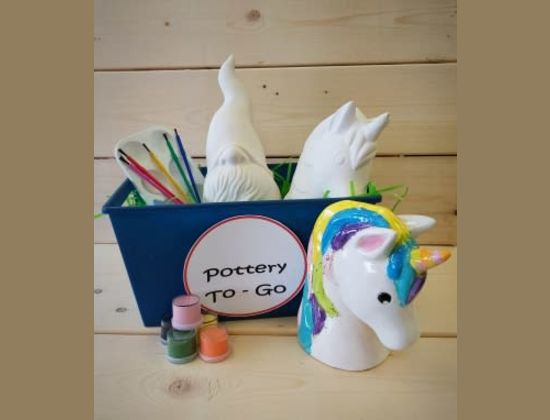# Take Home KitsCrafty Fox Studio At Home
, Bayonne, NJ

At-Home Kit

Crafty Fox Studio is officially a Mobile Studio! We are currently working on building our online store so in the meantime we will be using this order form for our Take Home Kits. Please read the following information carefully before placing your order.

• ​Placing an Order:  The order form is divided into sections to better organize all the pieces we…

### Party Animals/Tots/Figurines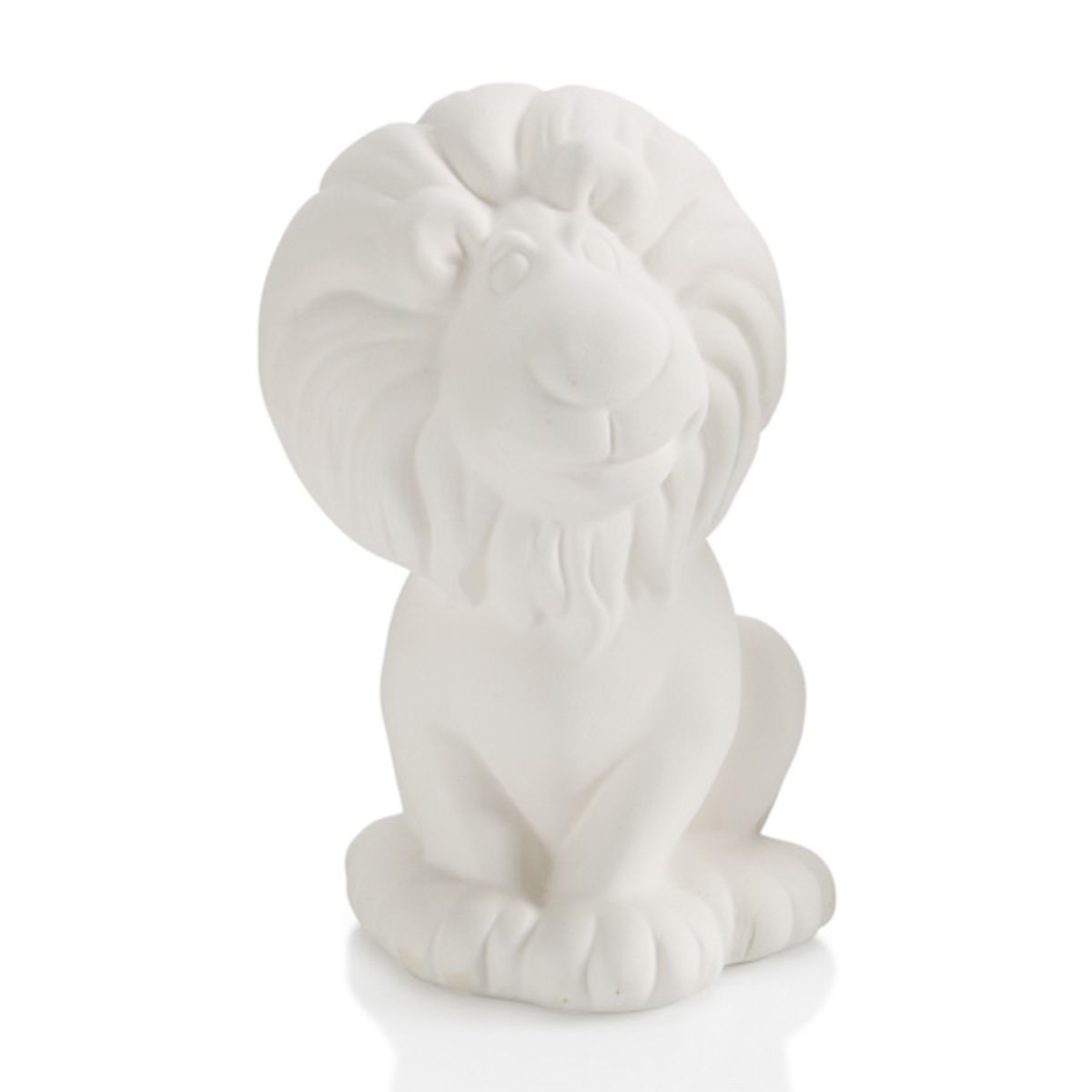(Max of 1)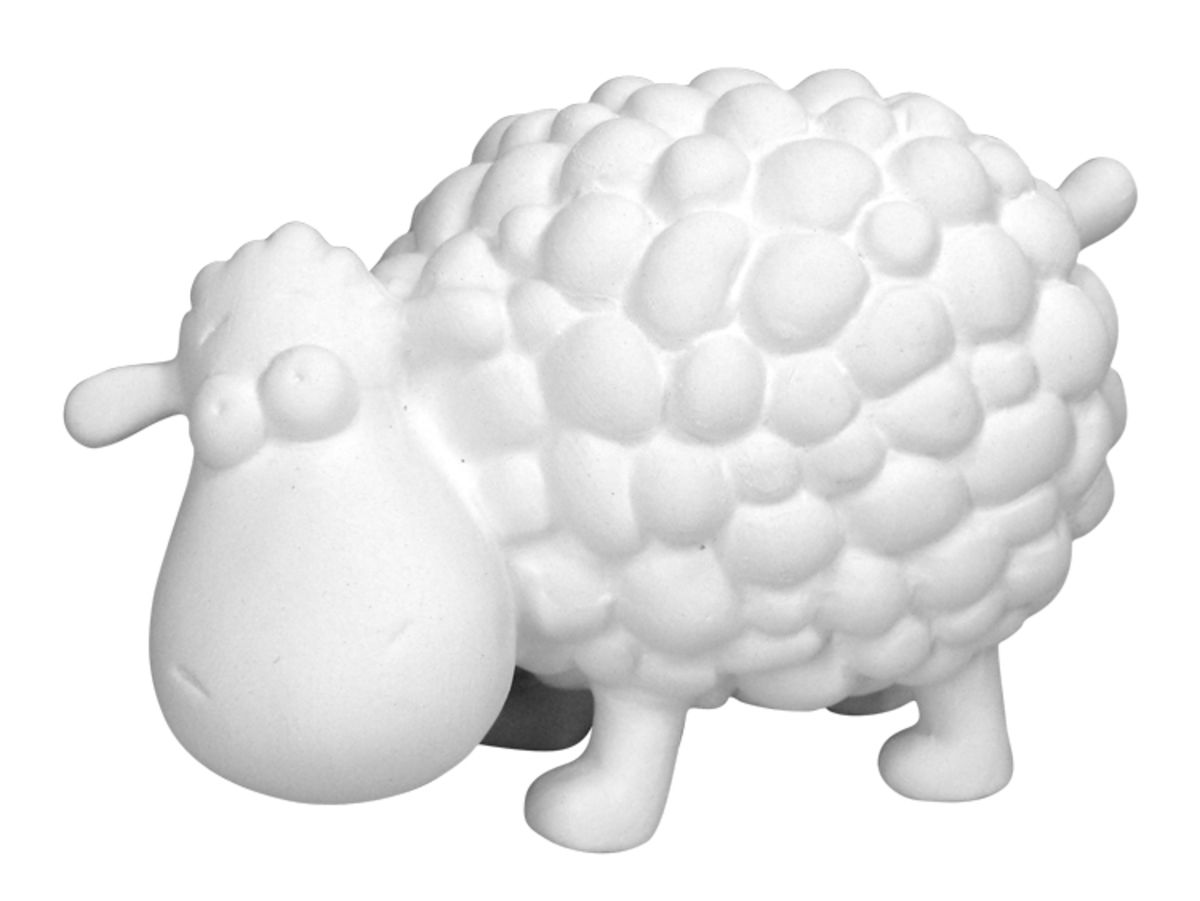(Max of 3)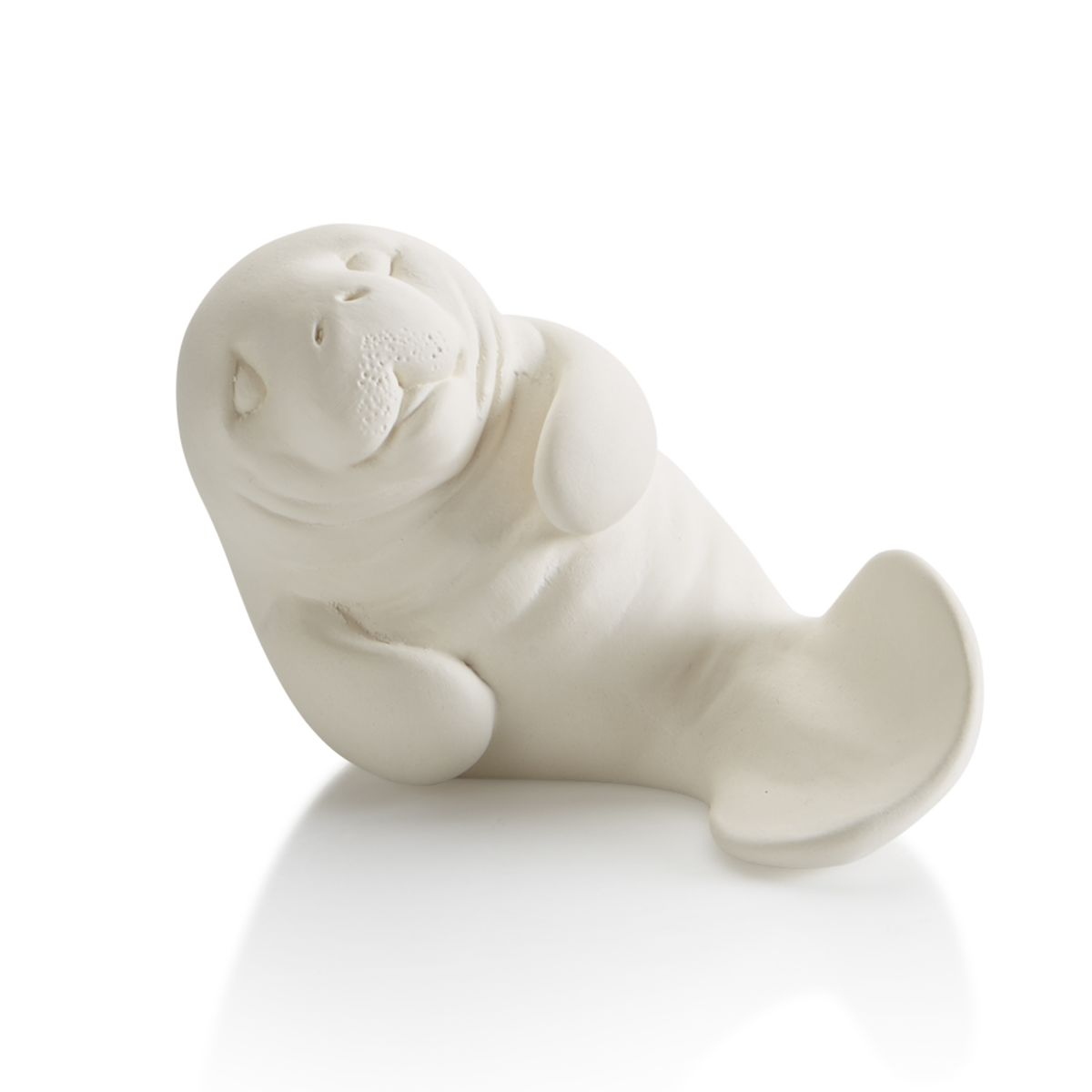(Max of 2)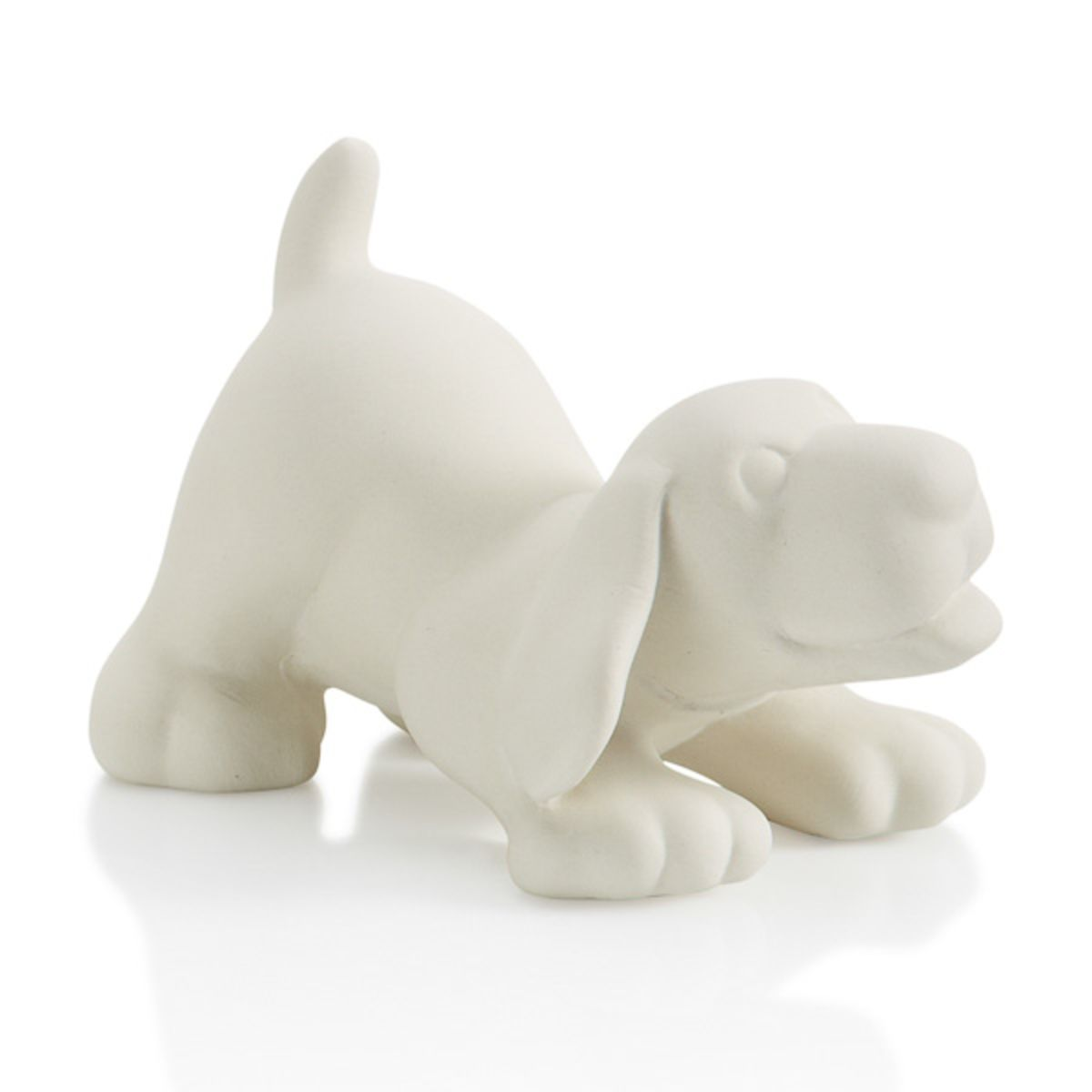(Max of 1)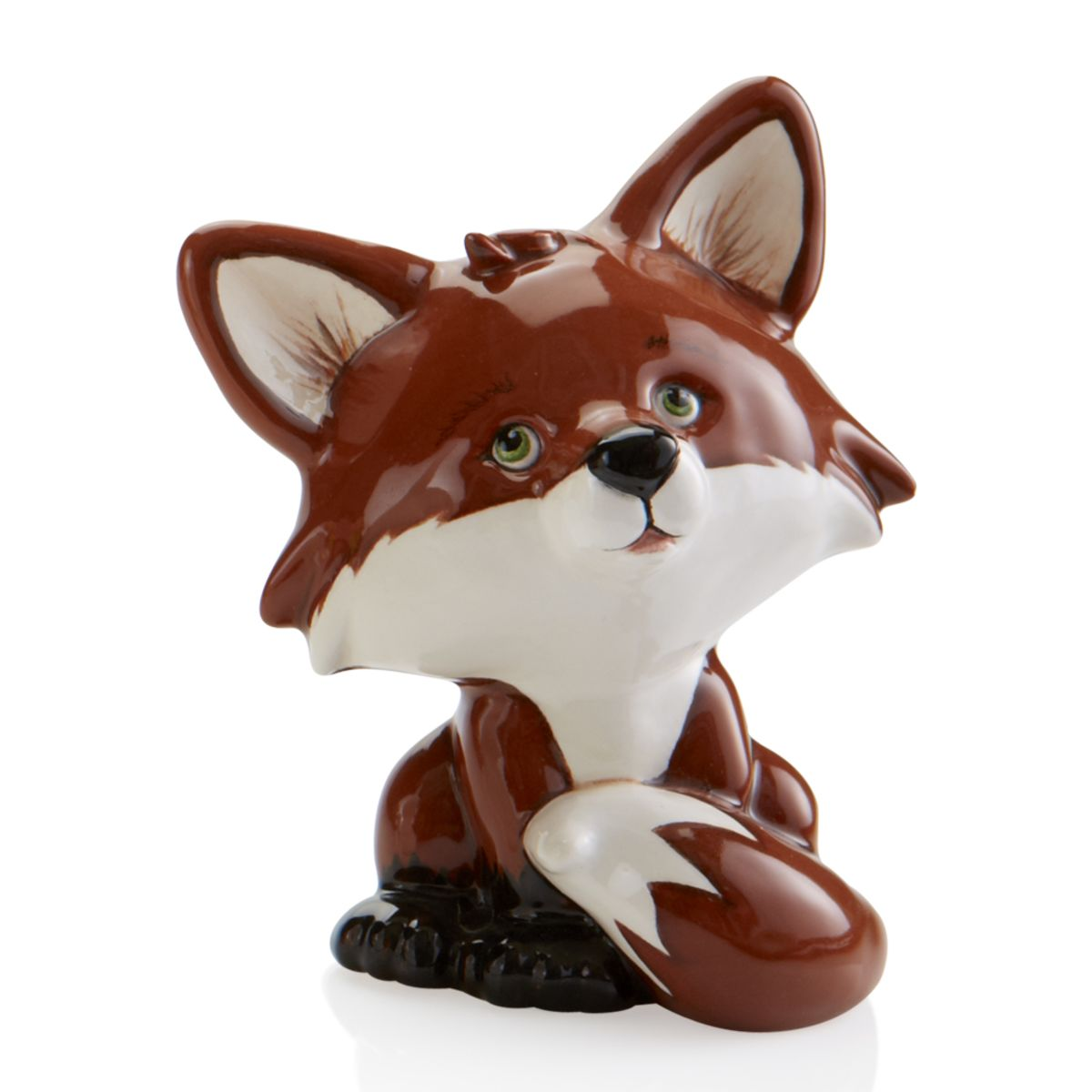(Max of 8)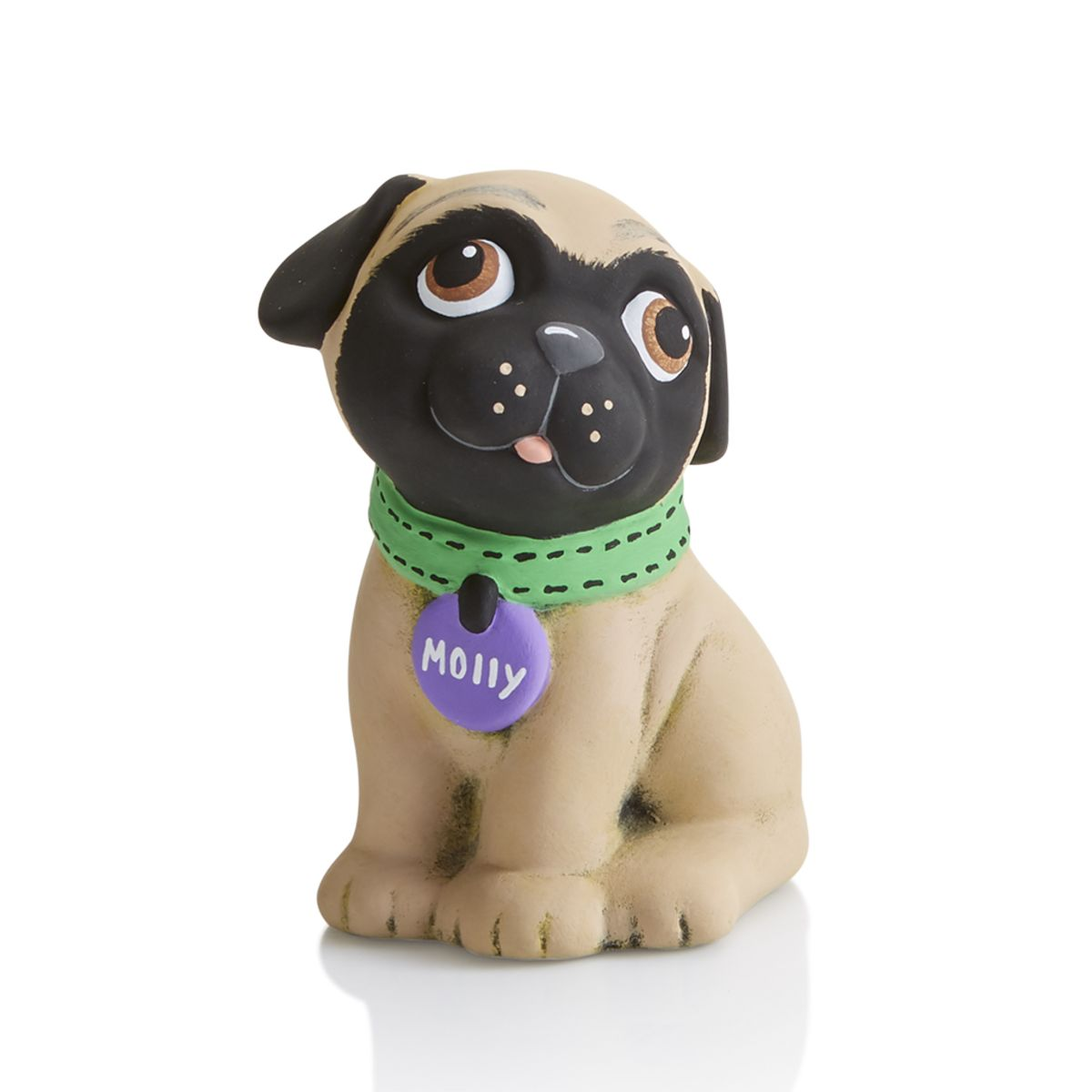(Max of 8)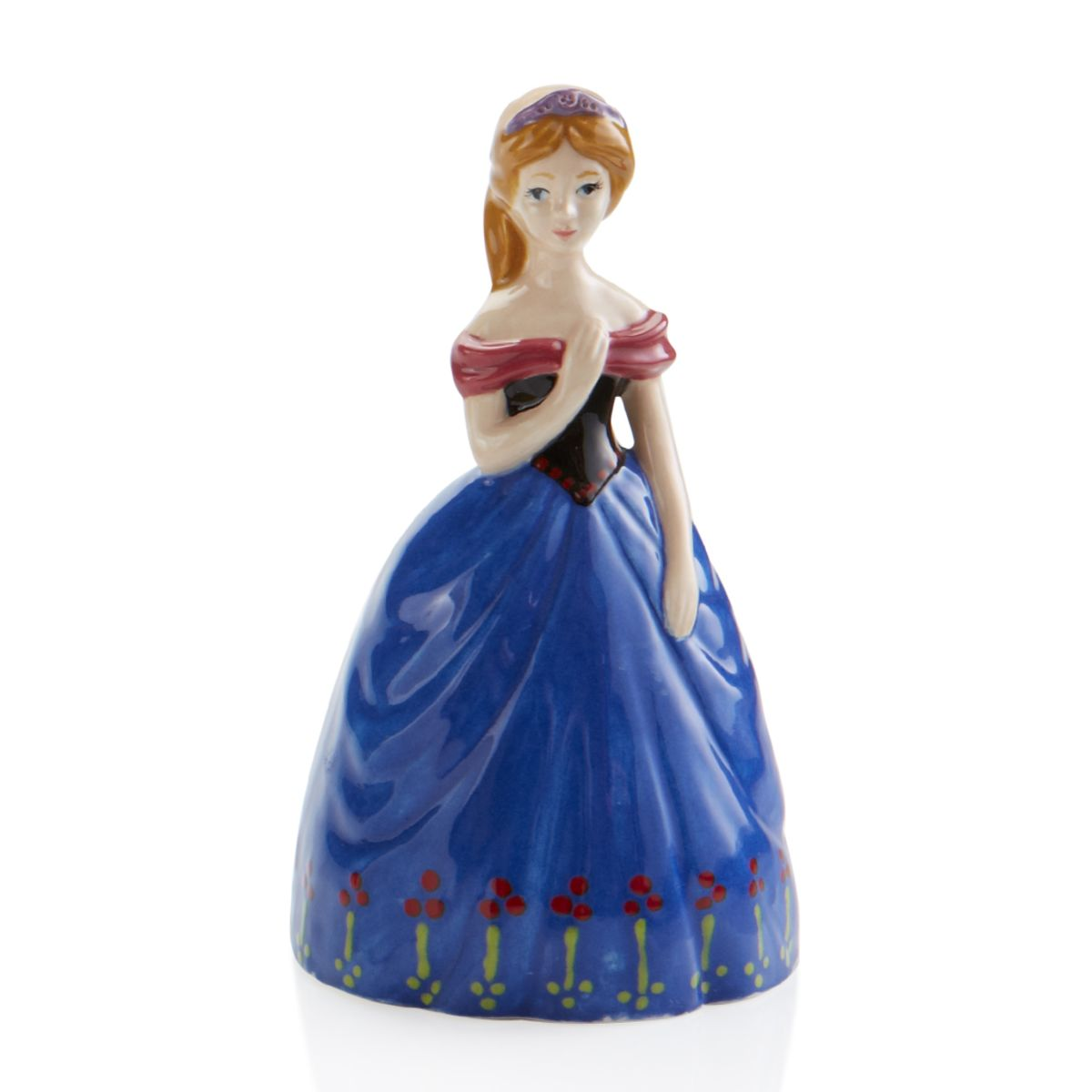(Max of 8)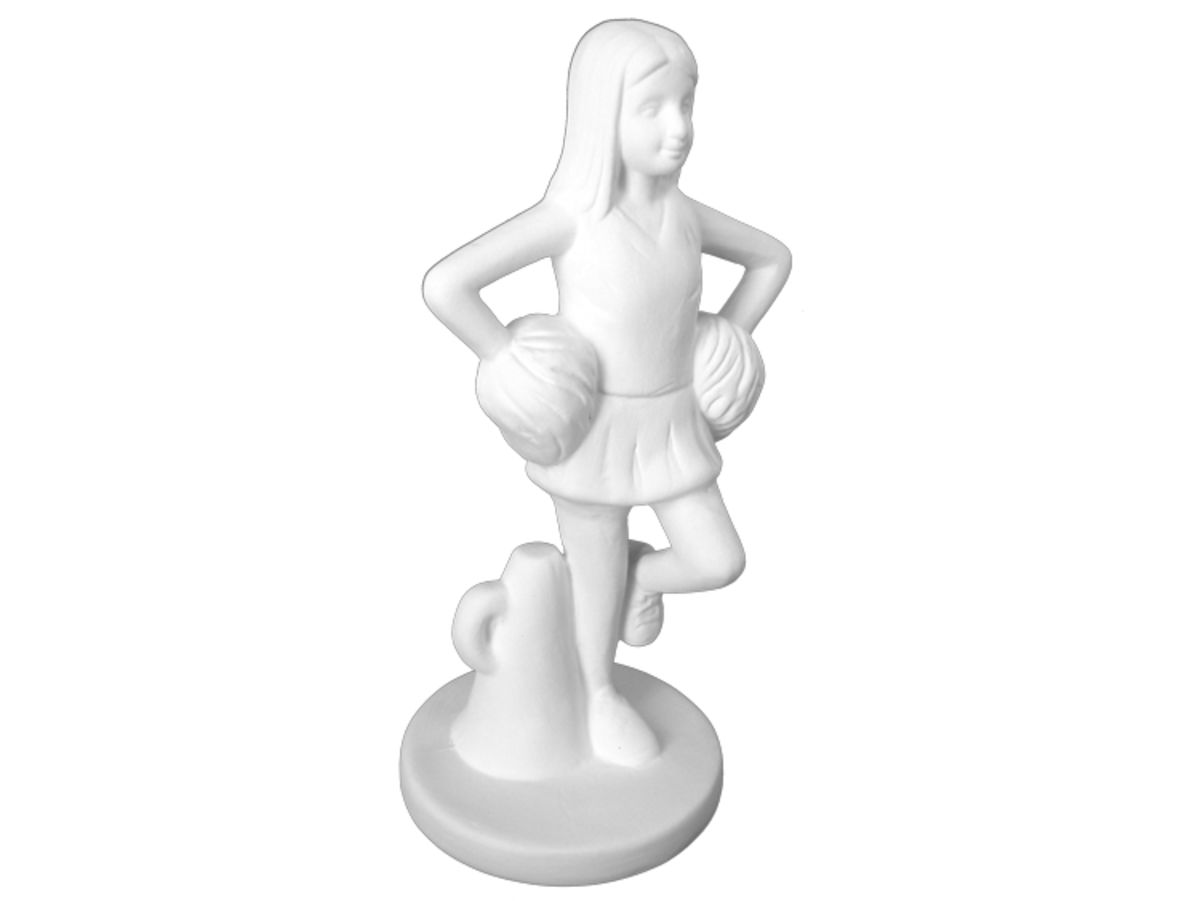(Max of 2)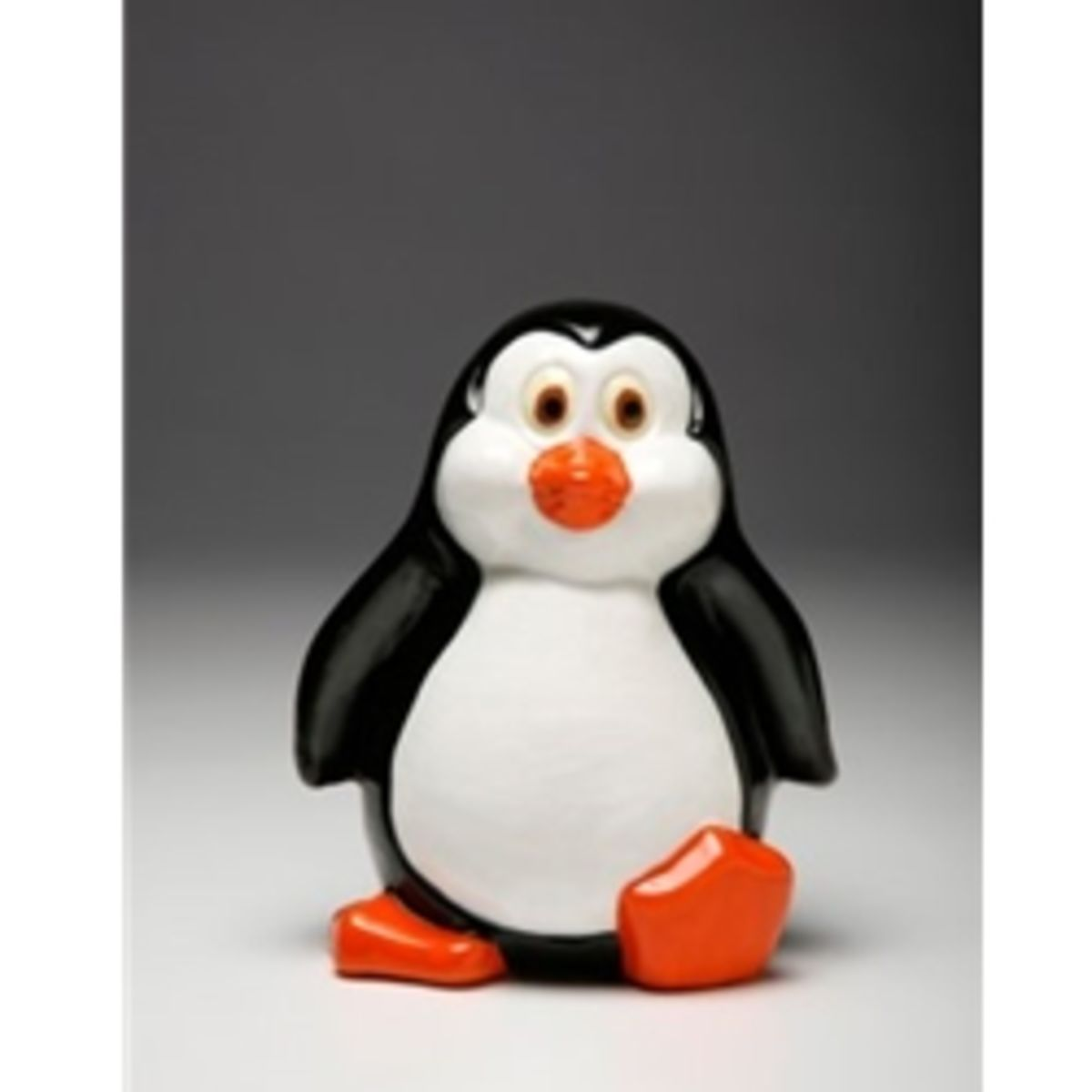(Max of 4)(Max of 3)

### Holiday Pieces(Max of 2)(Max of 1)(Max of 4)(Max of 1)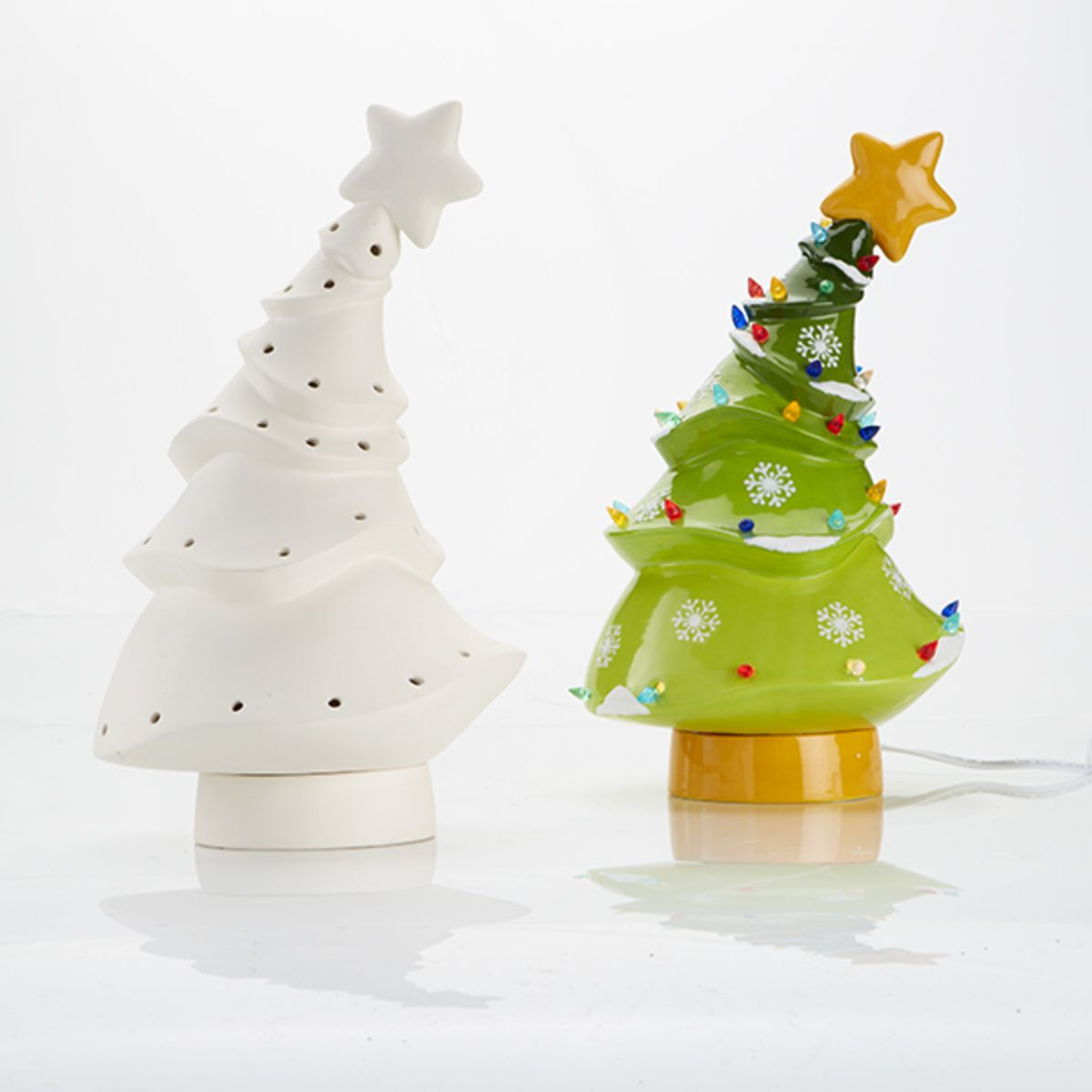(Max of 3)

### Color Me in Pottery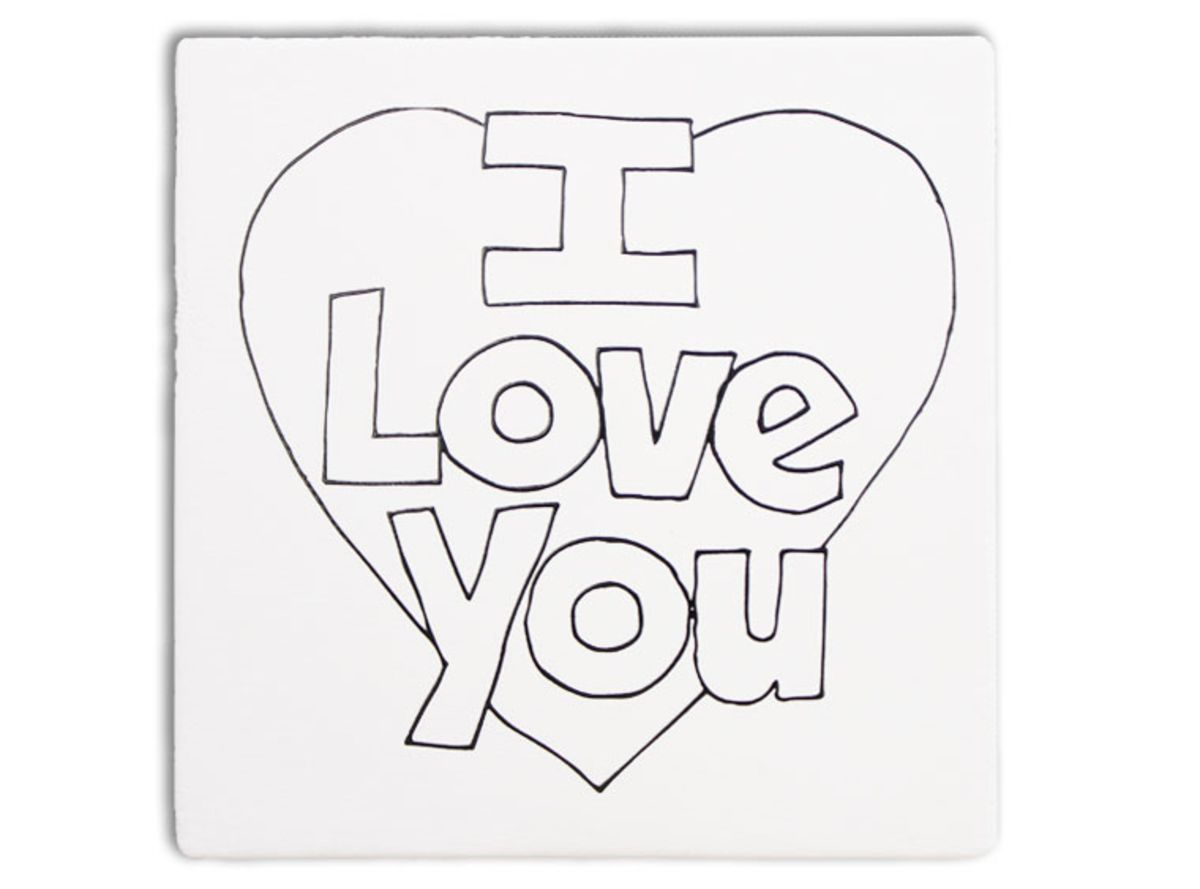(Max of 1)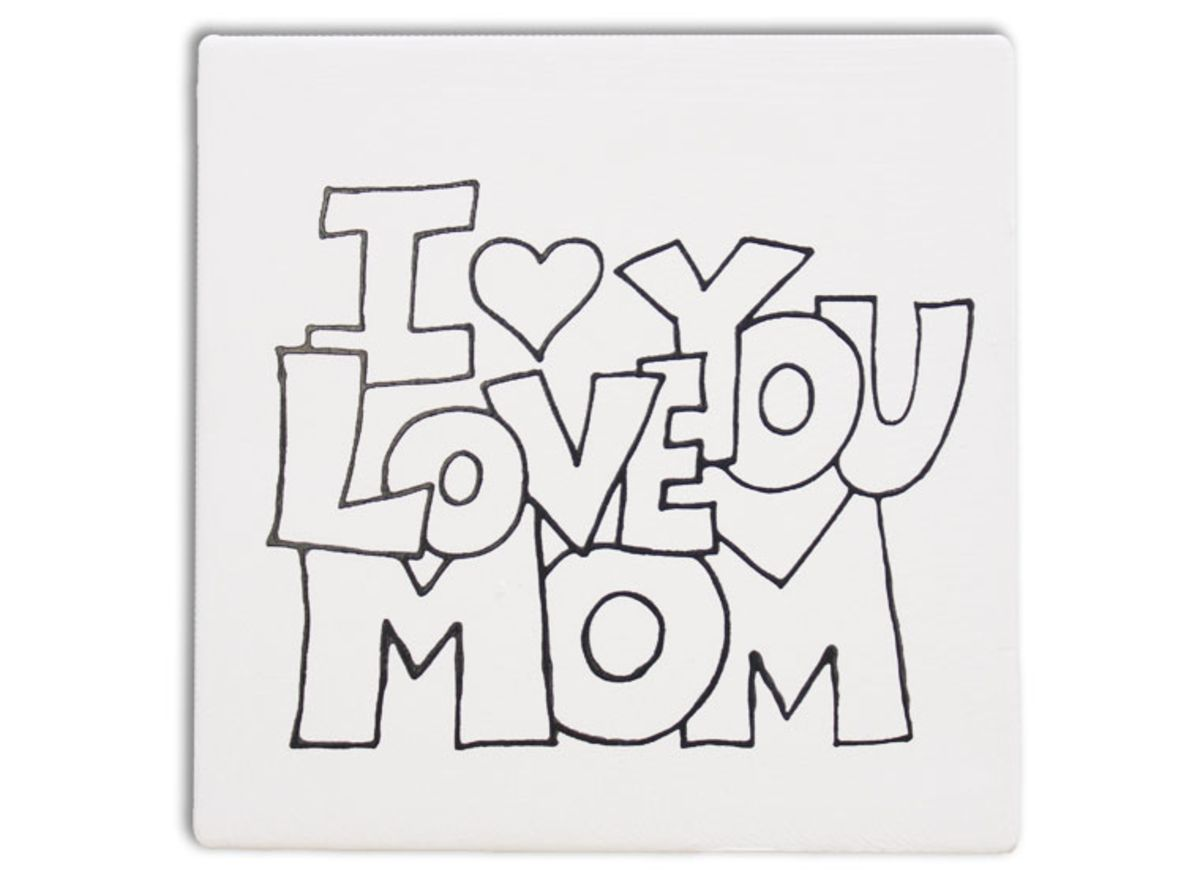(Max of 4)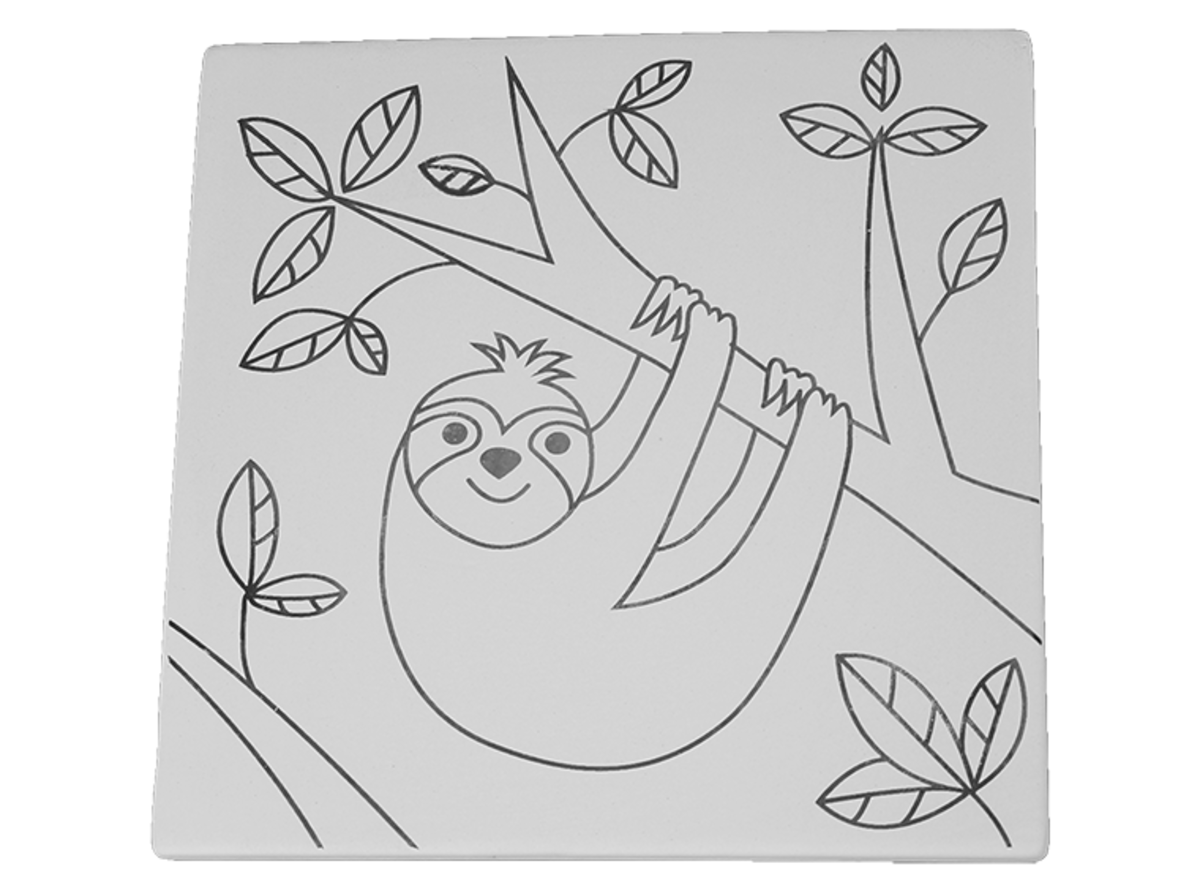(Max of 11)

### Star Wars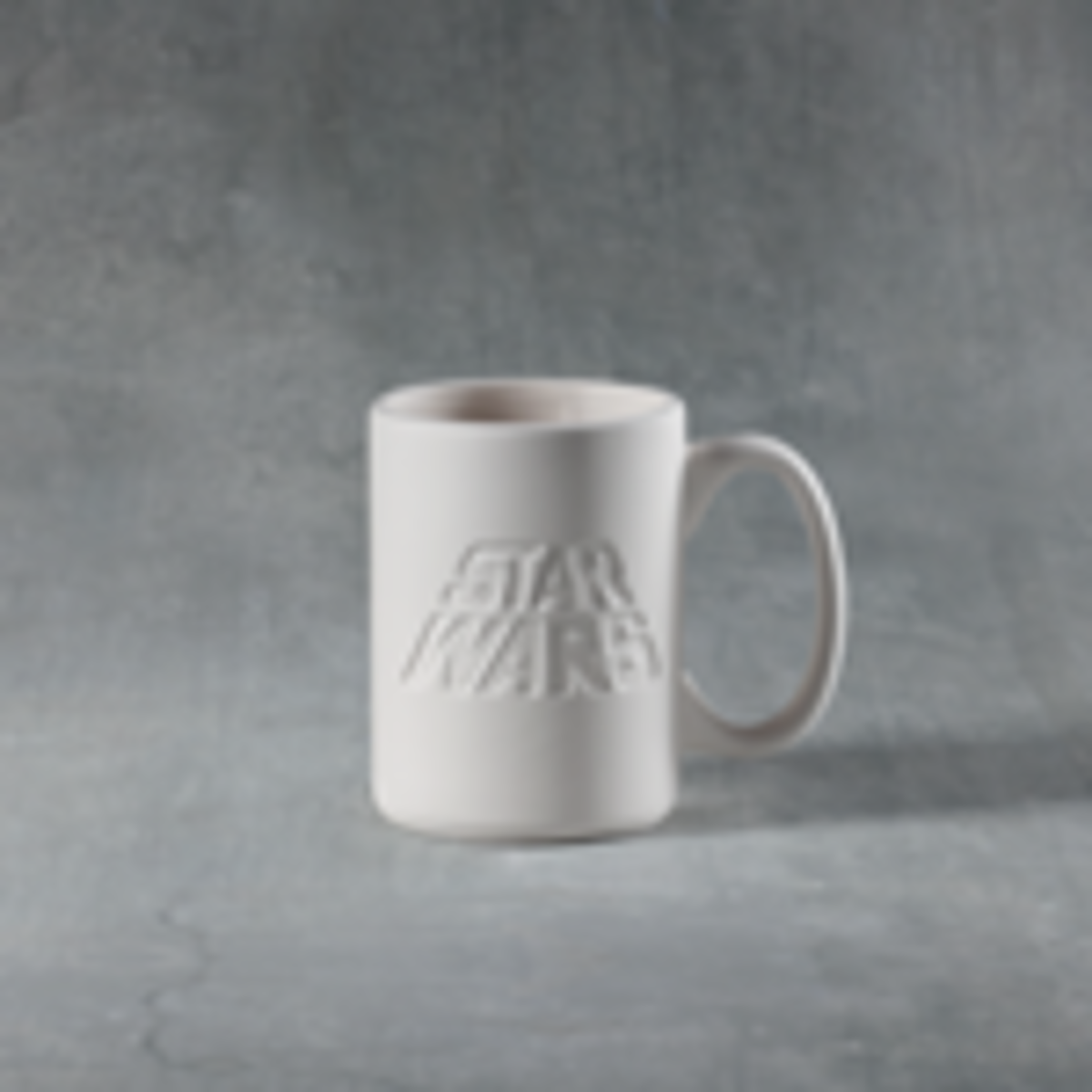(Max of 6)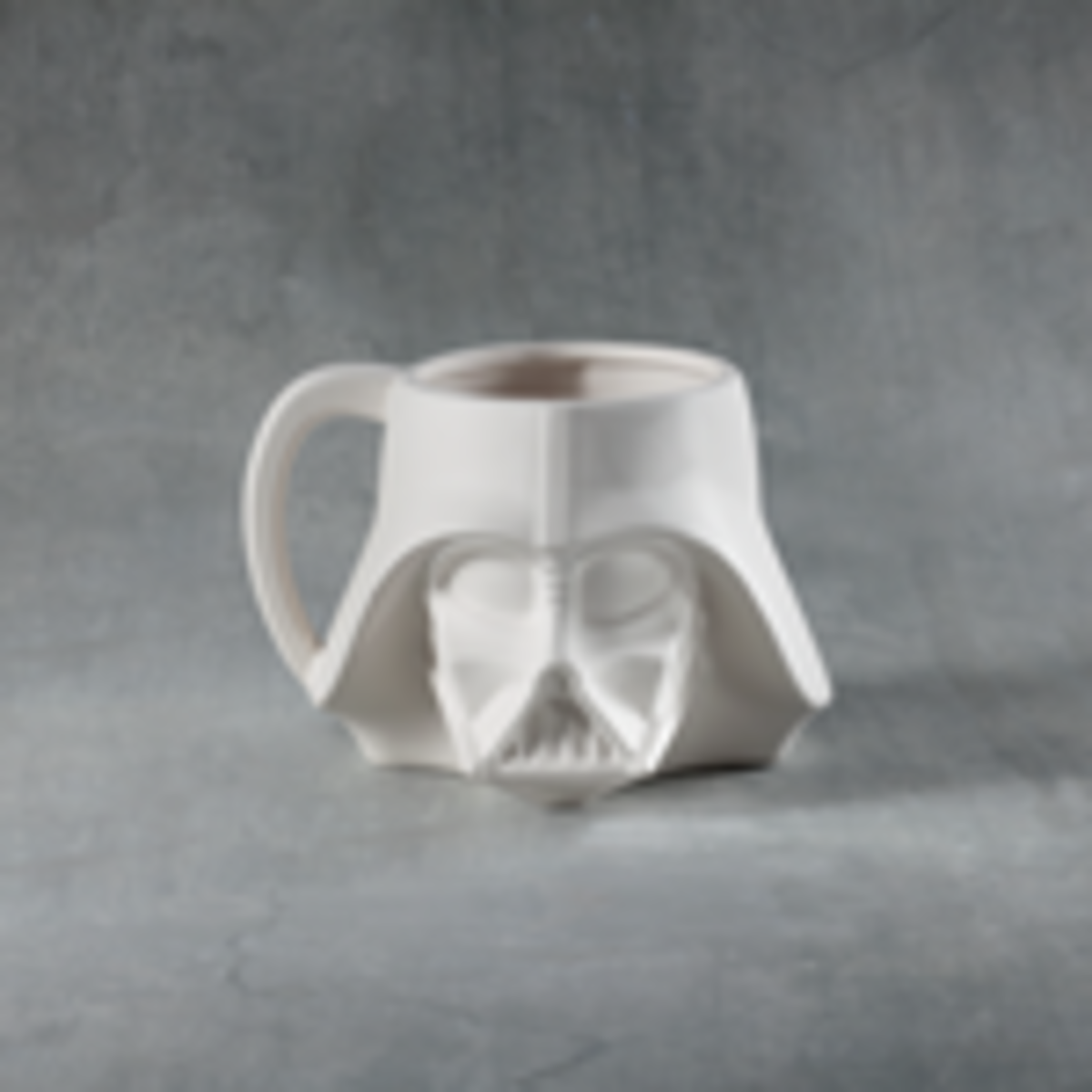(Max of 6)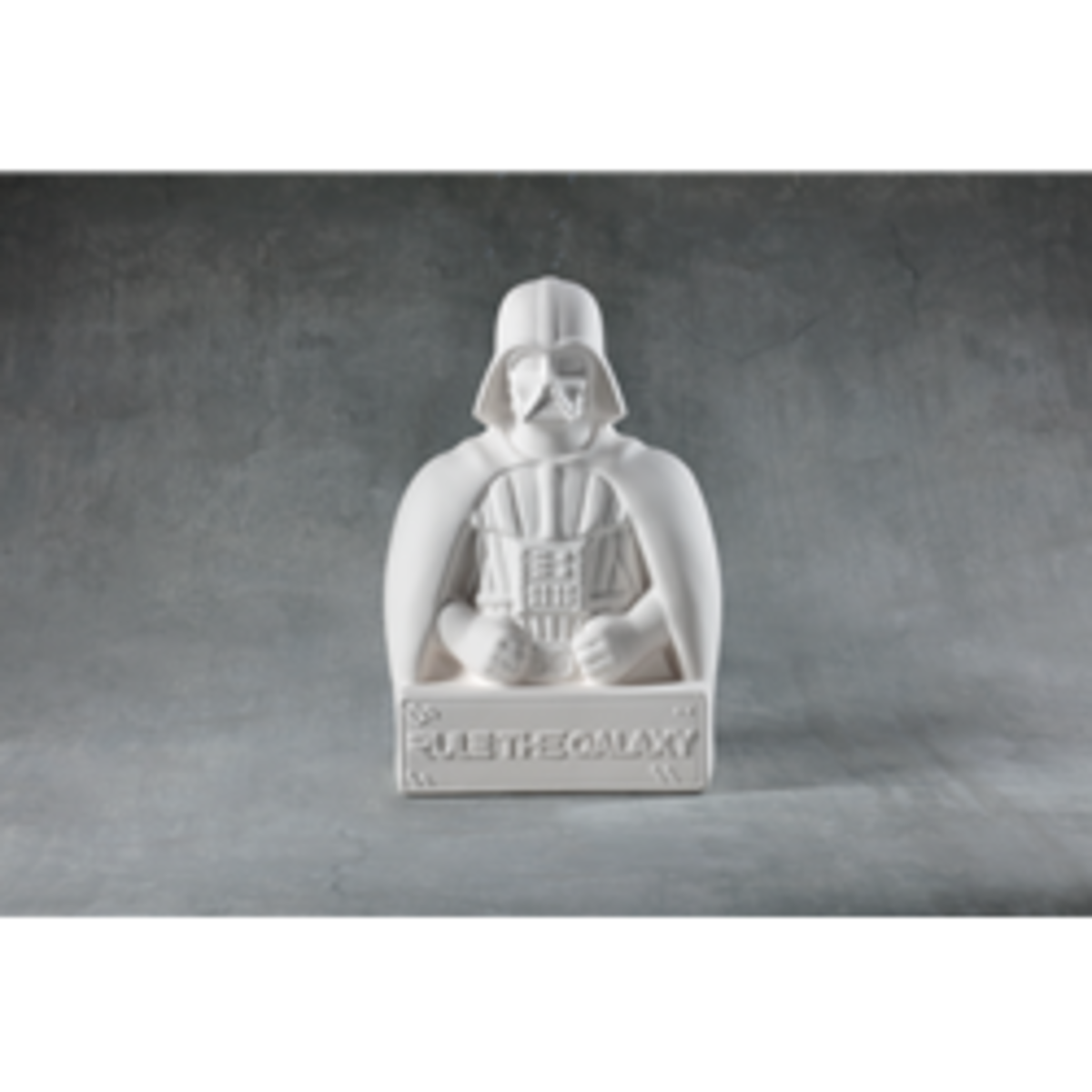(Max of 6)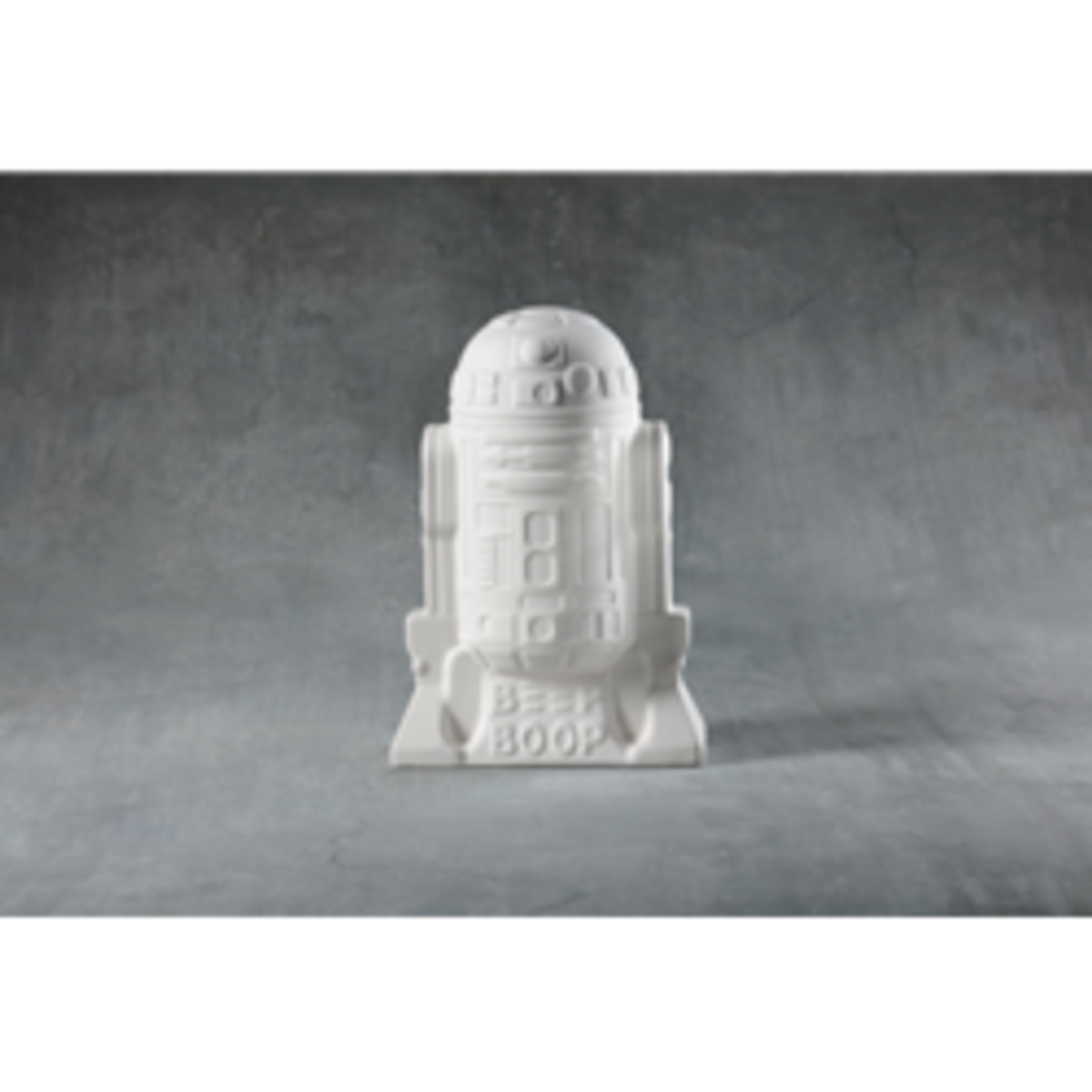(Max of 3)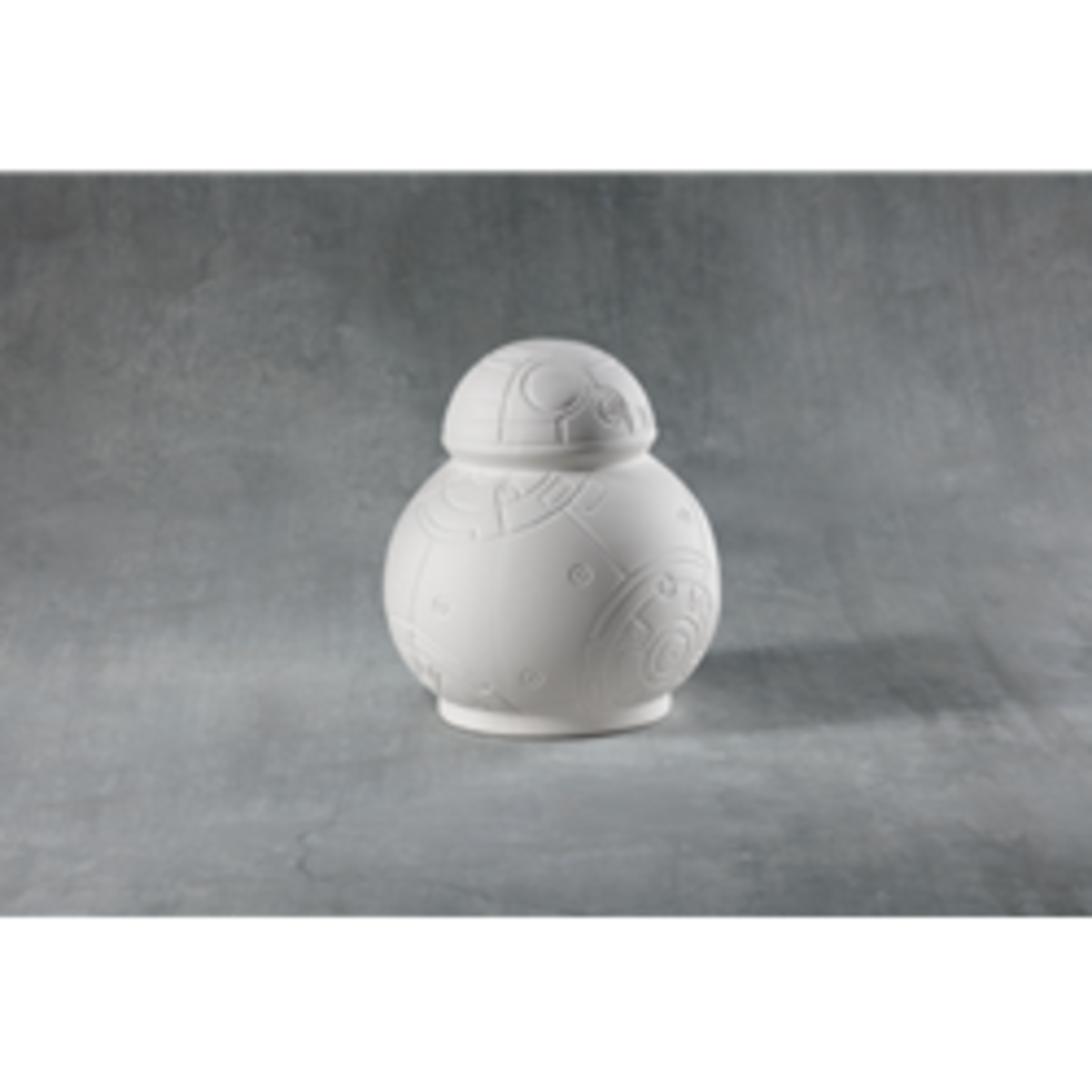(Max of 3)

### Banks and Boxes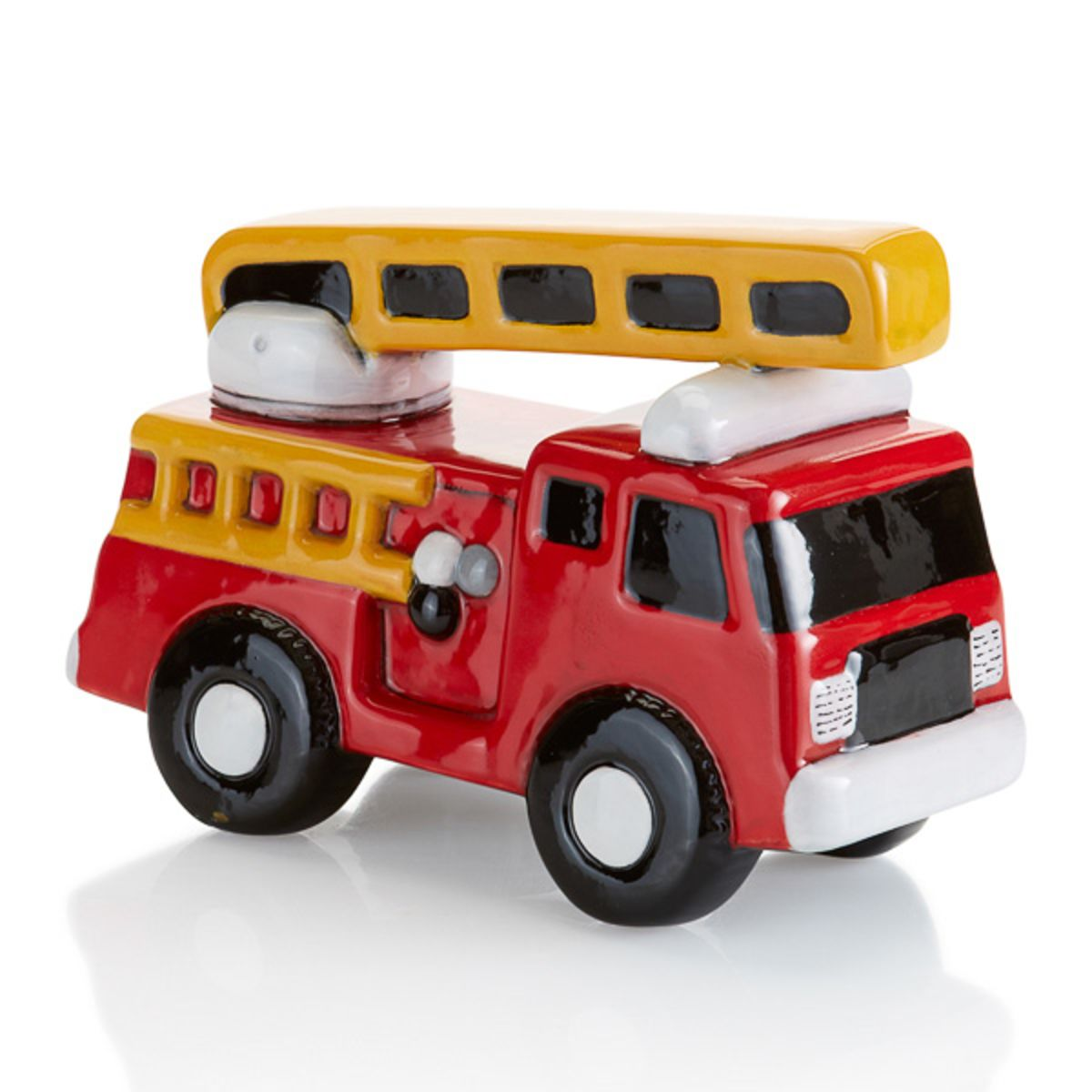(Max of 1)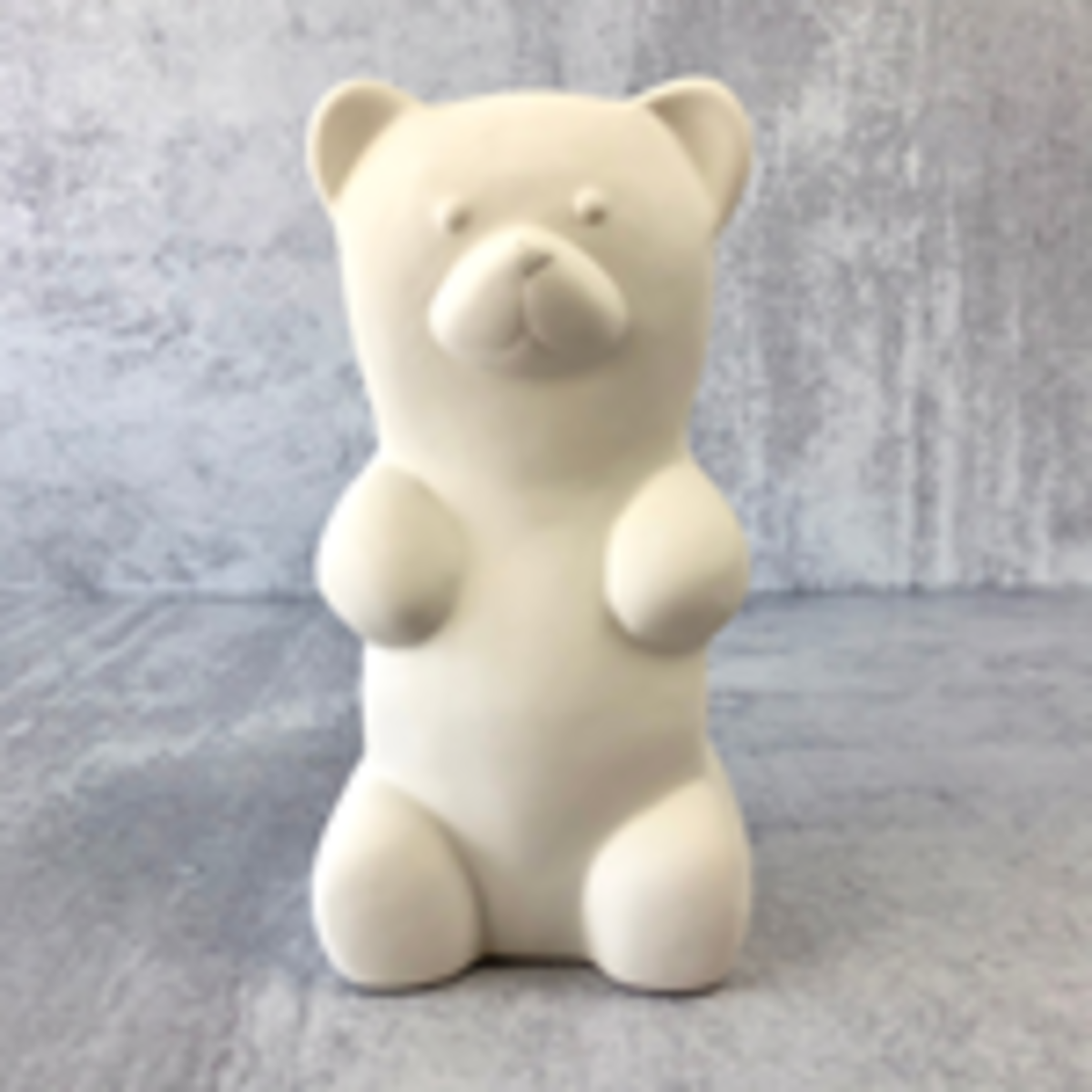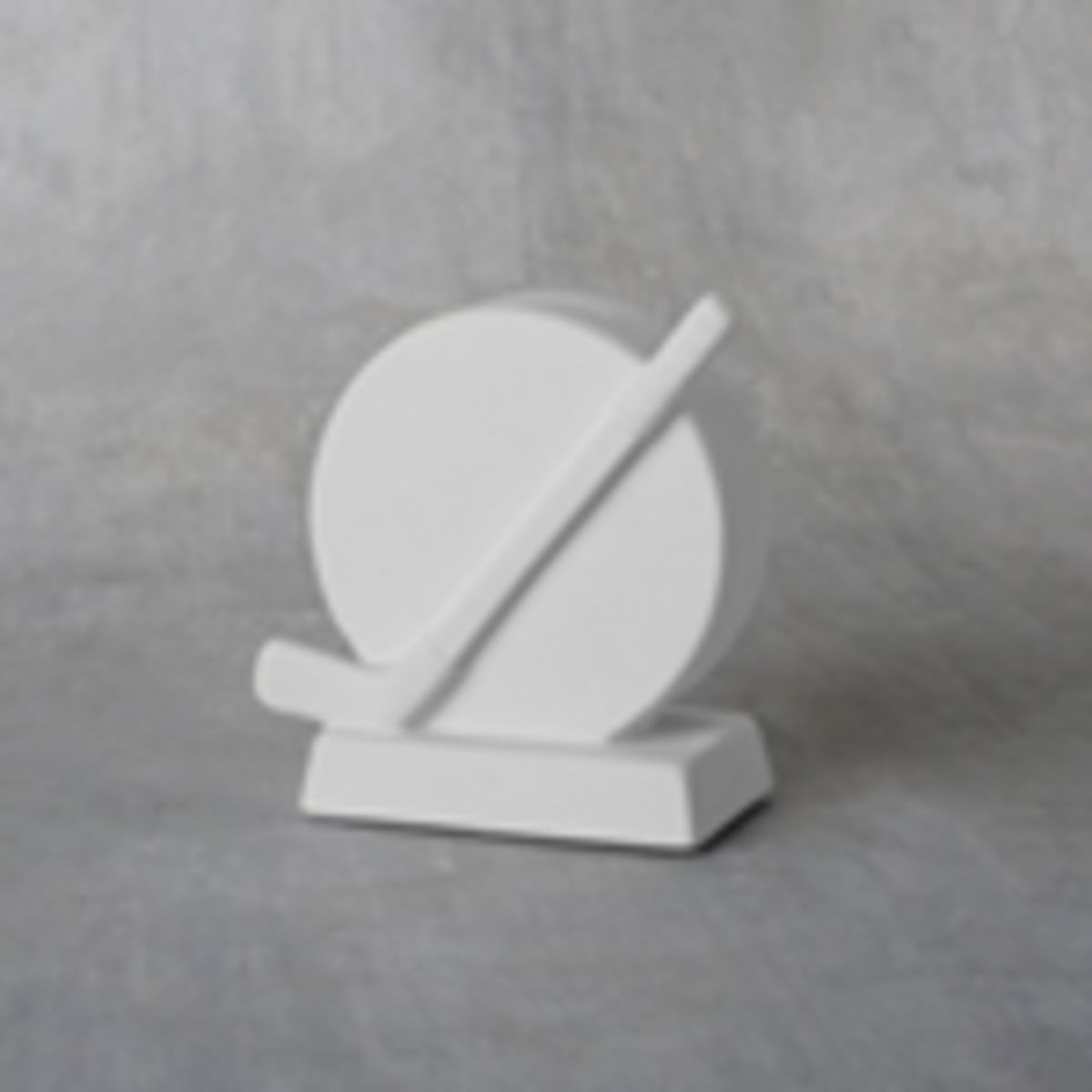(Max of 5)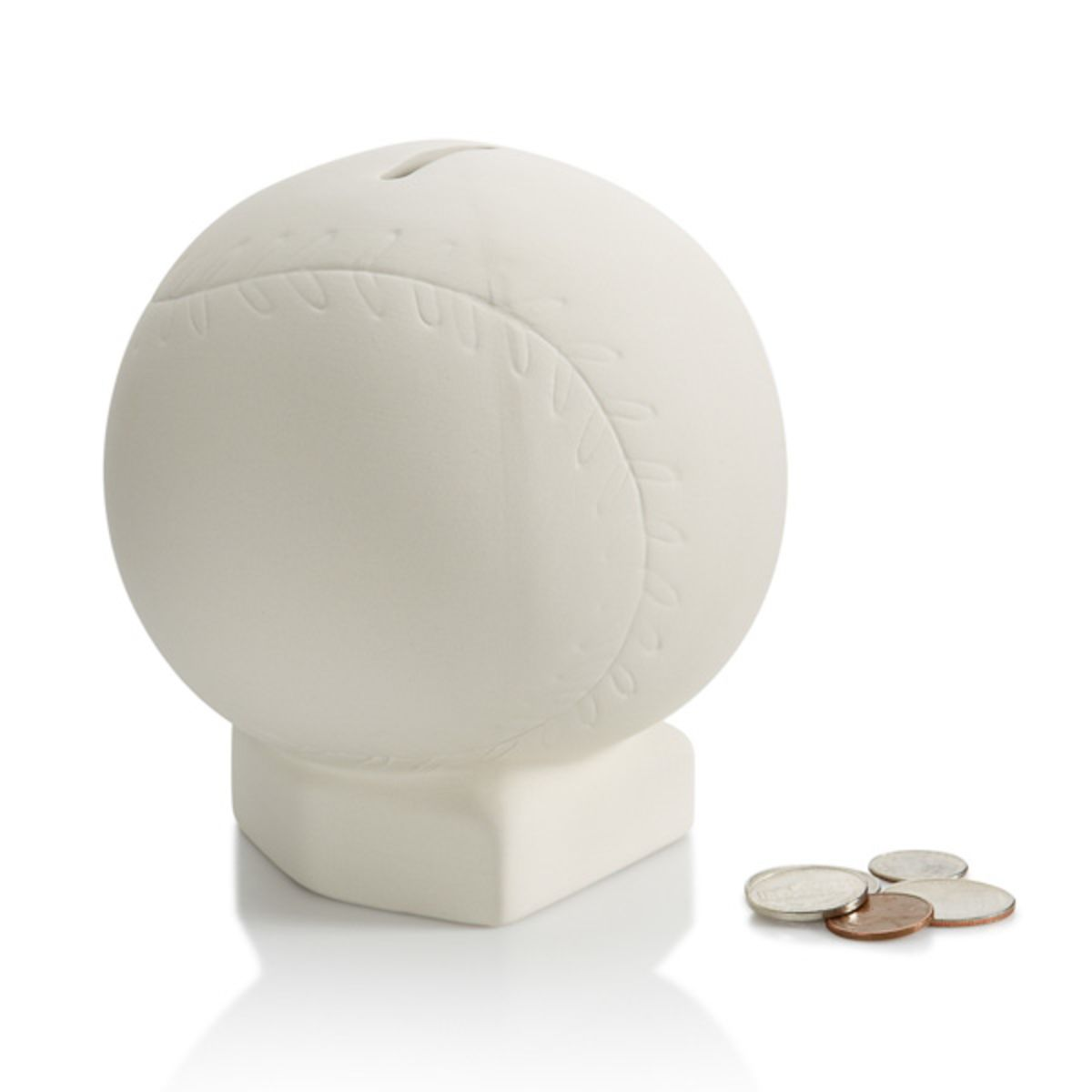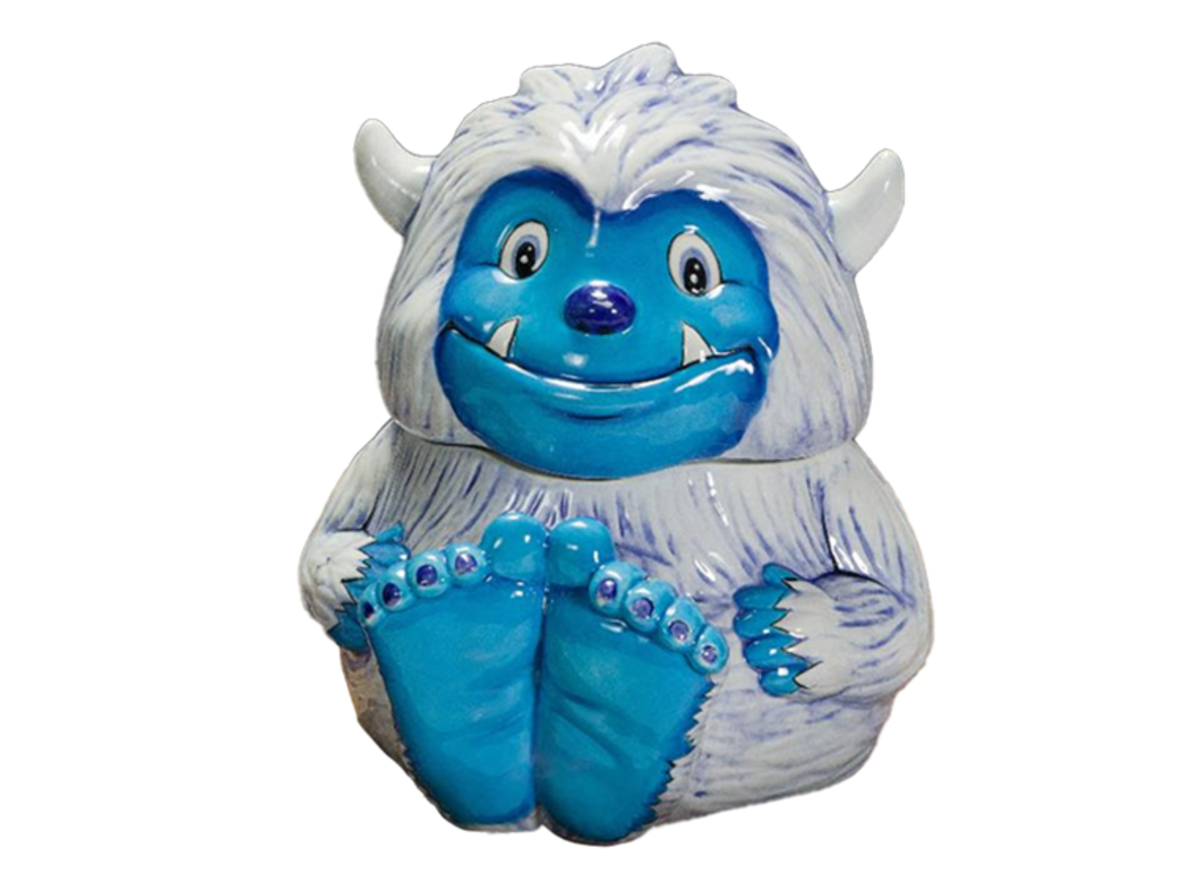(Max of 2)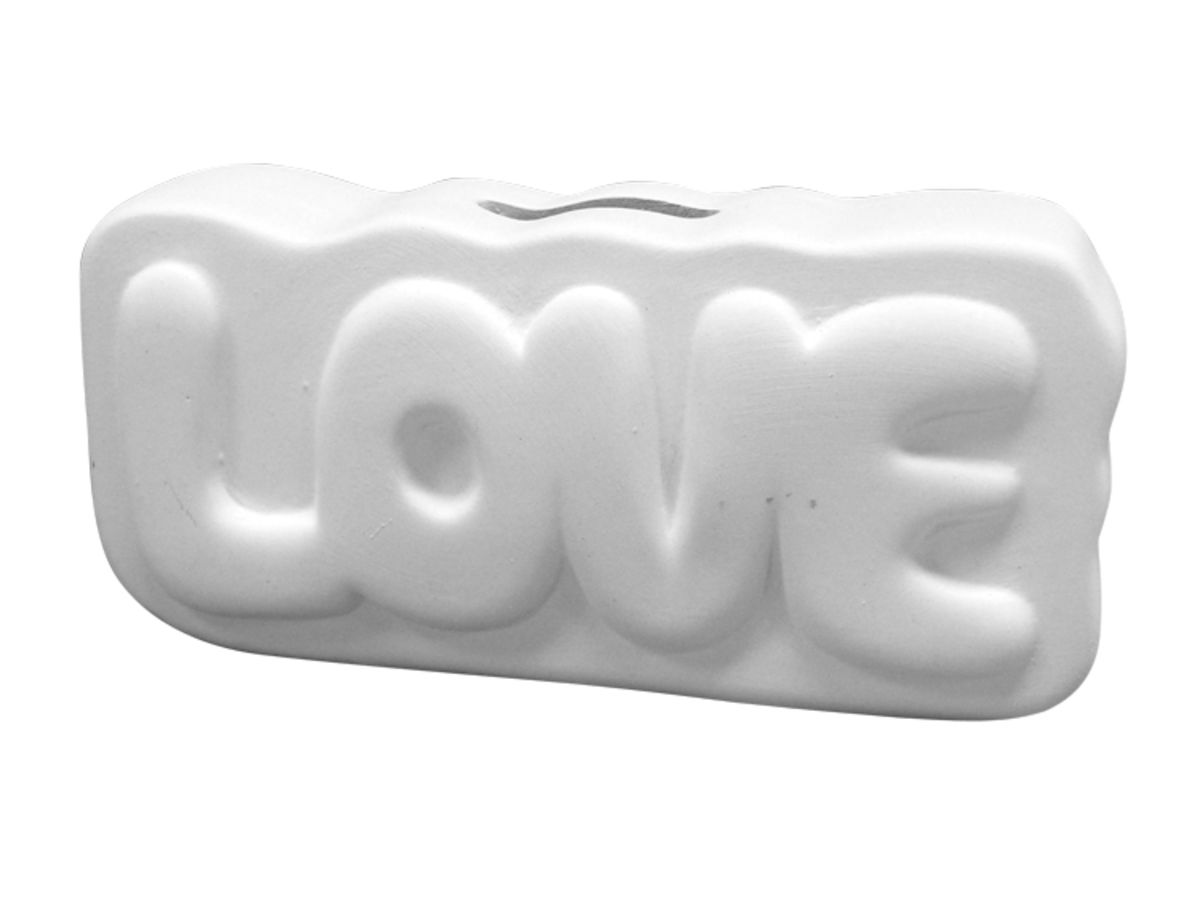(Max of 3)

### Home Decor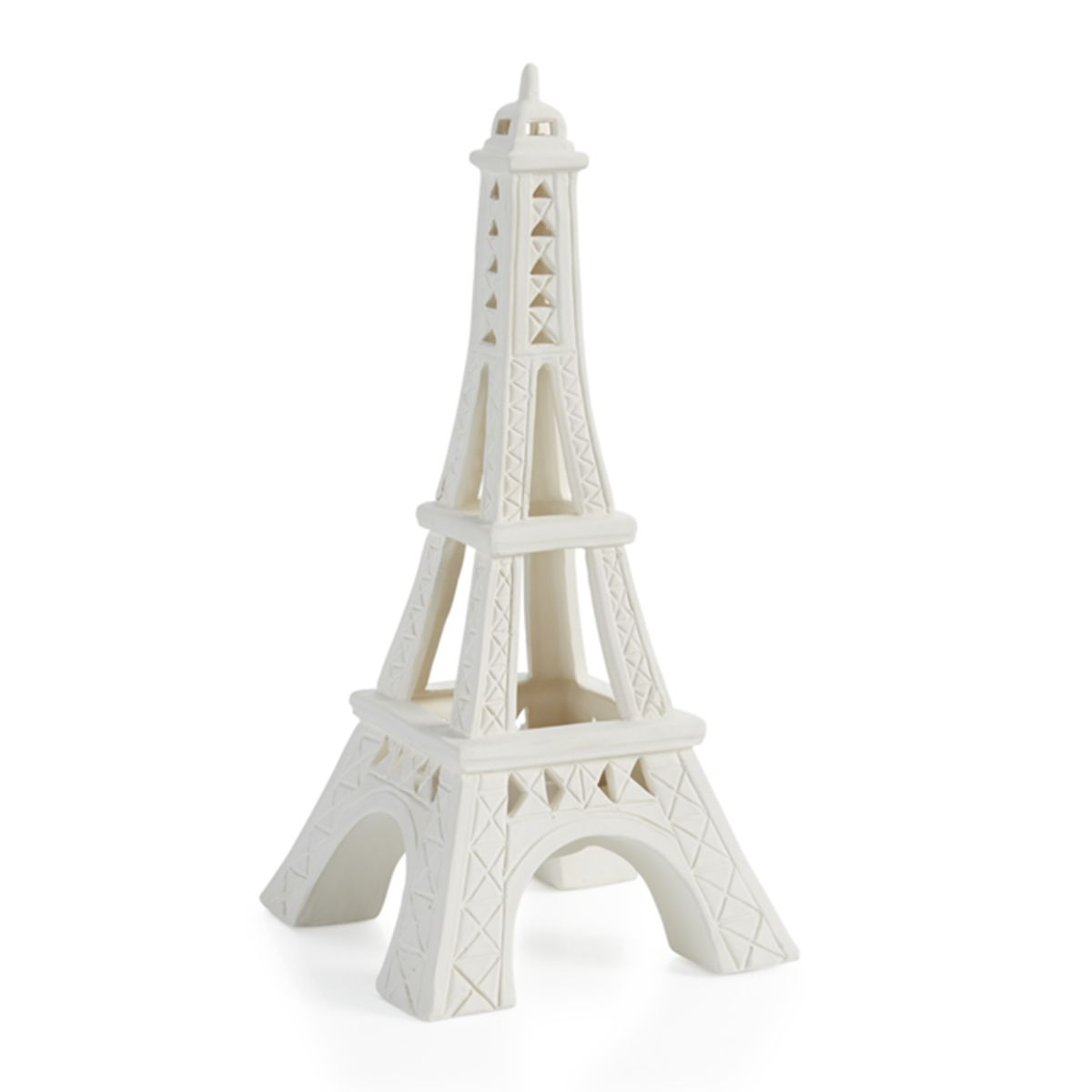(Max of 1)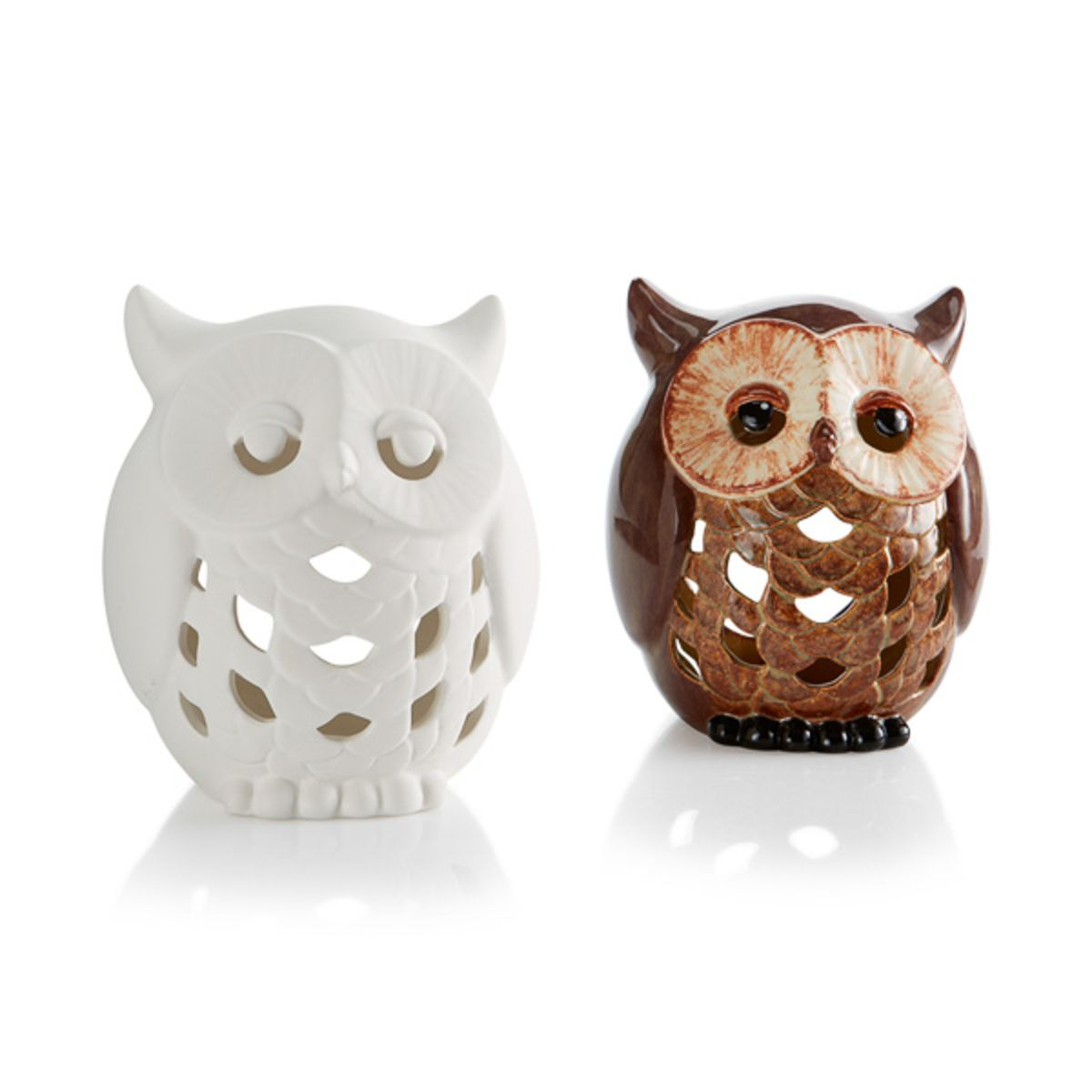(Max of 1)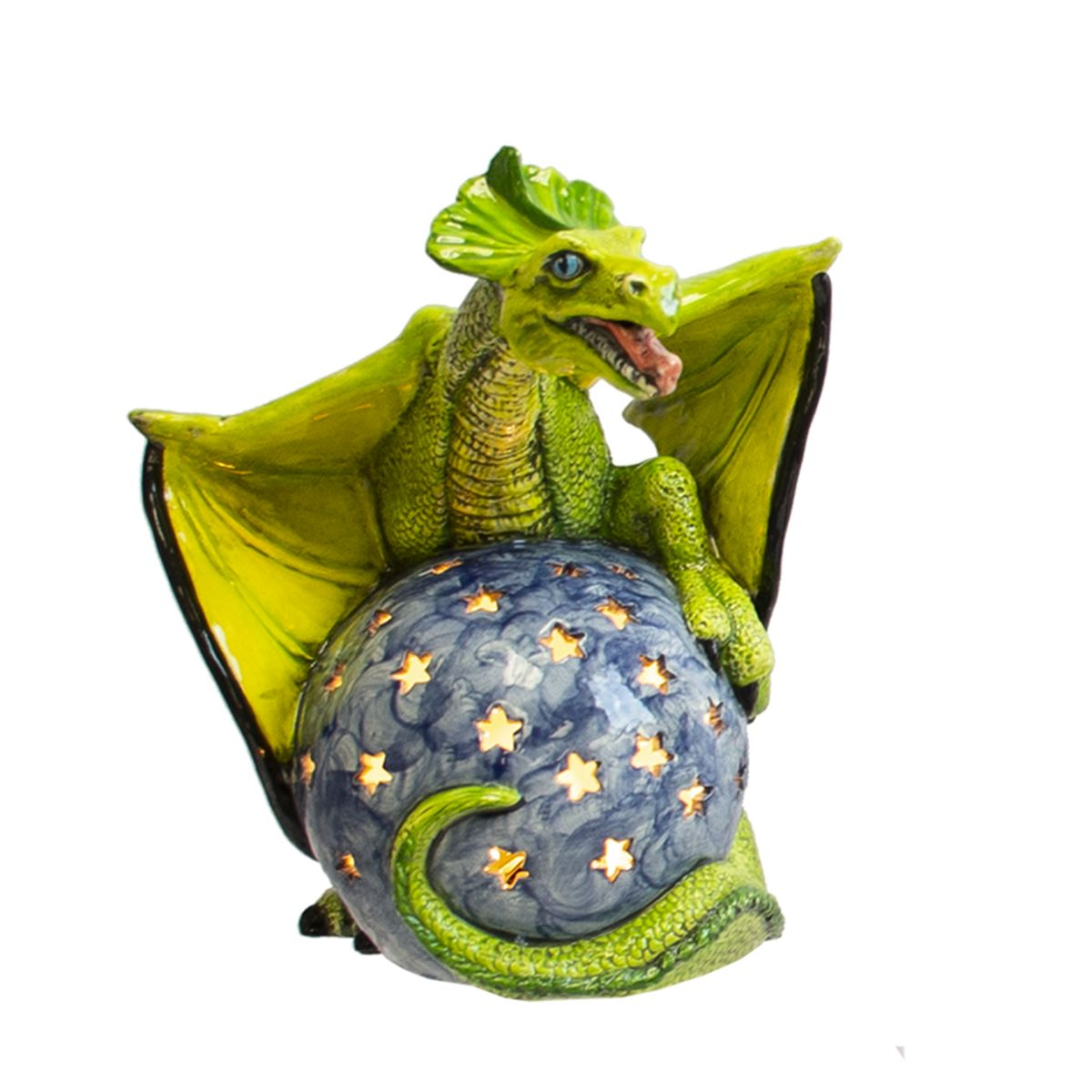(Max of 2)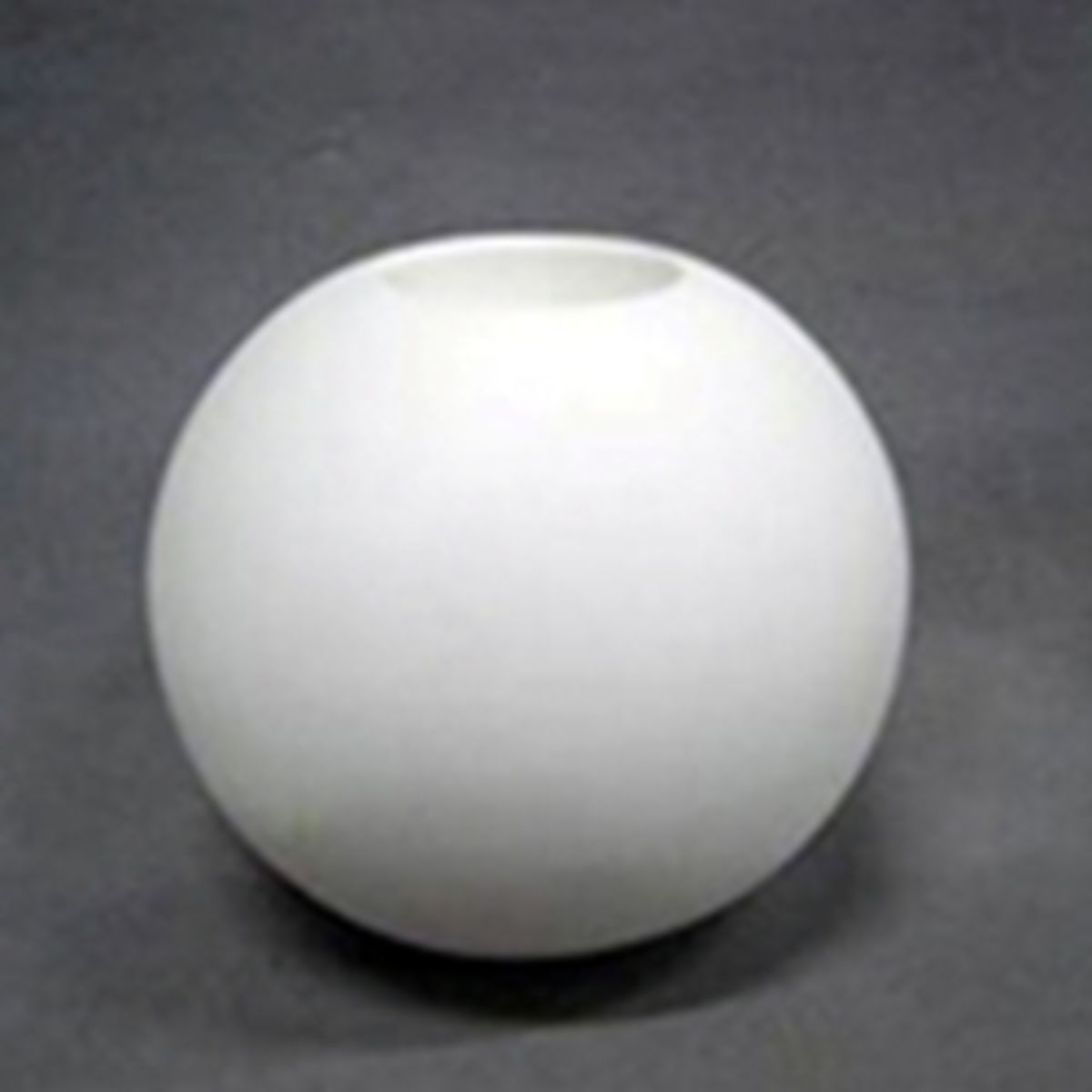(Max of 1)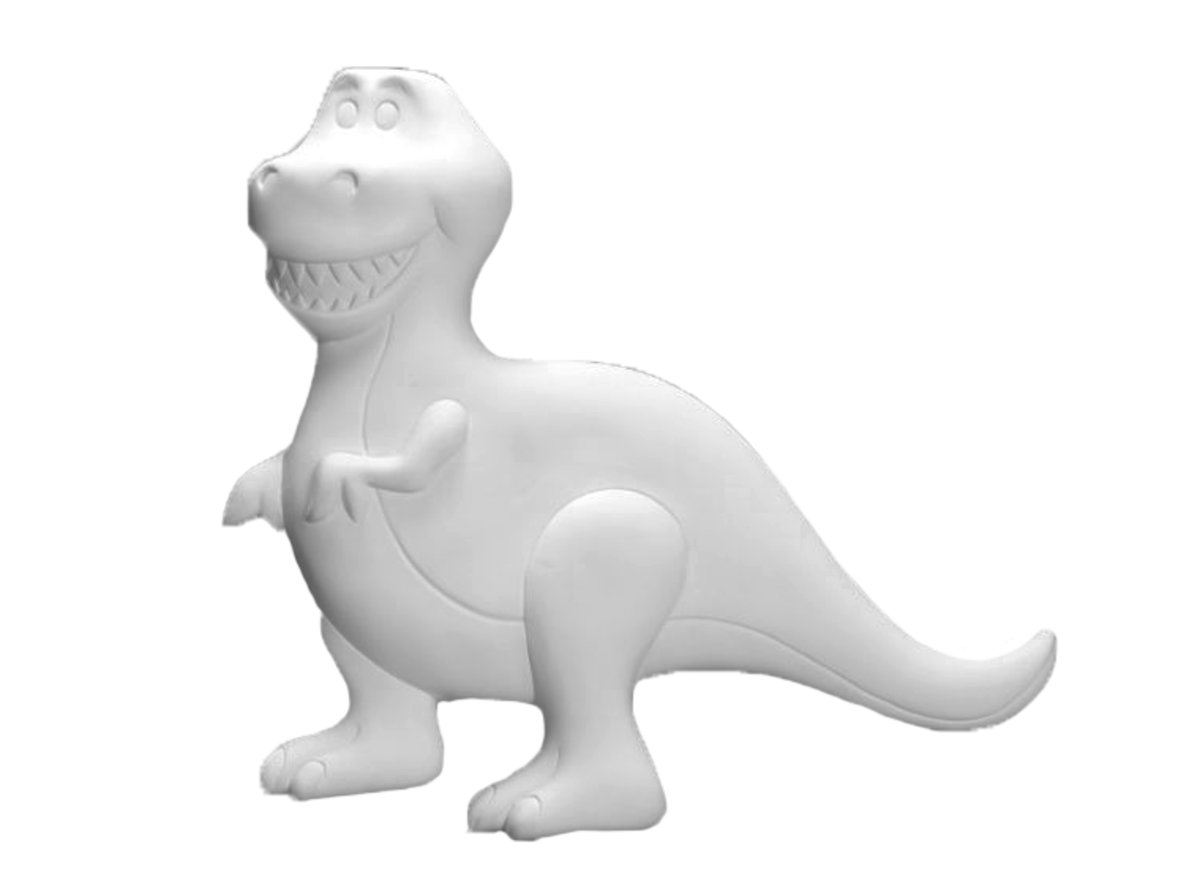(Max of 1)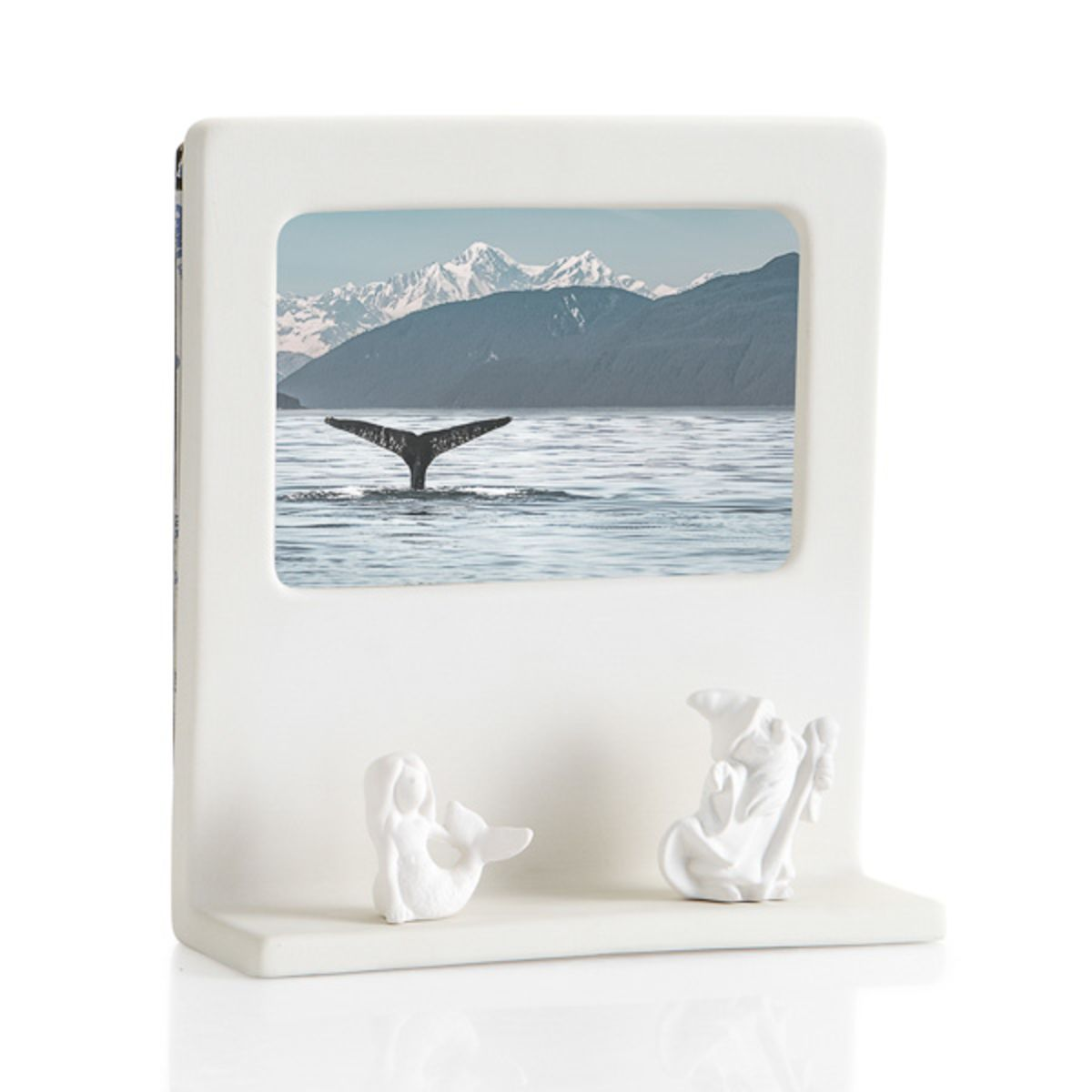(Max of 6)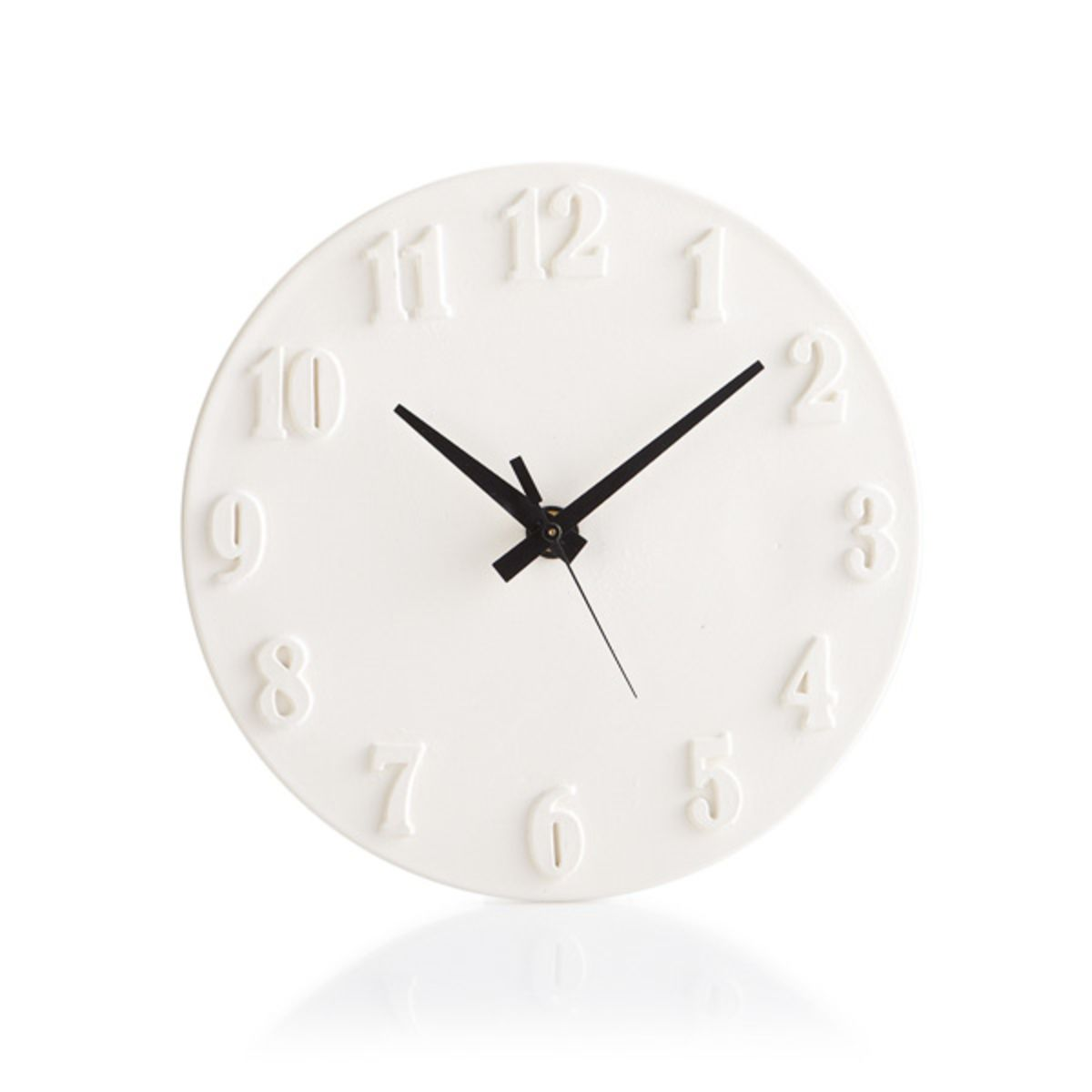(Max of 3)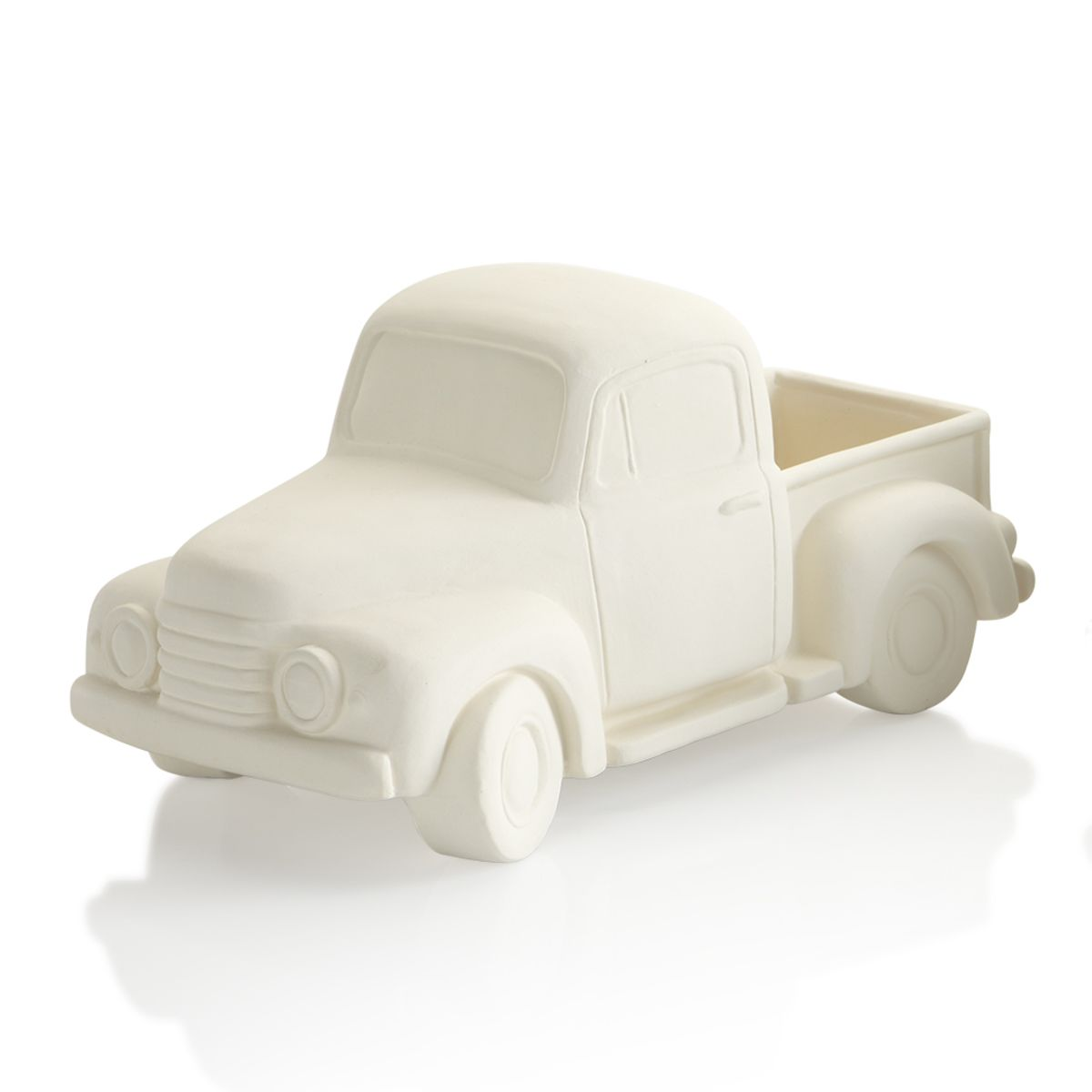(Max of 1)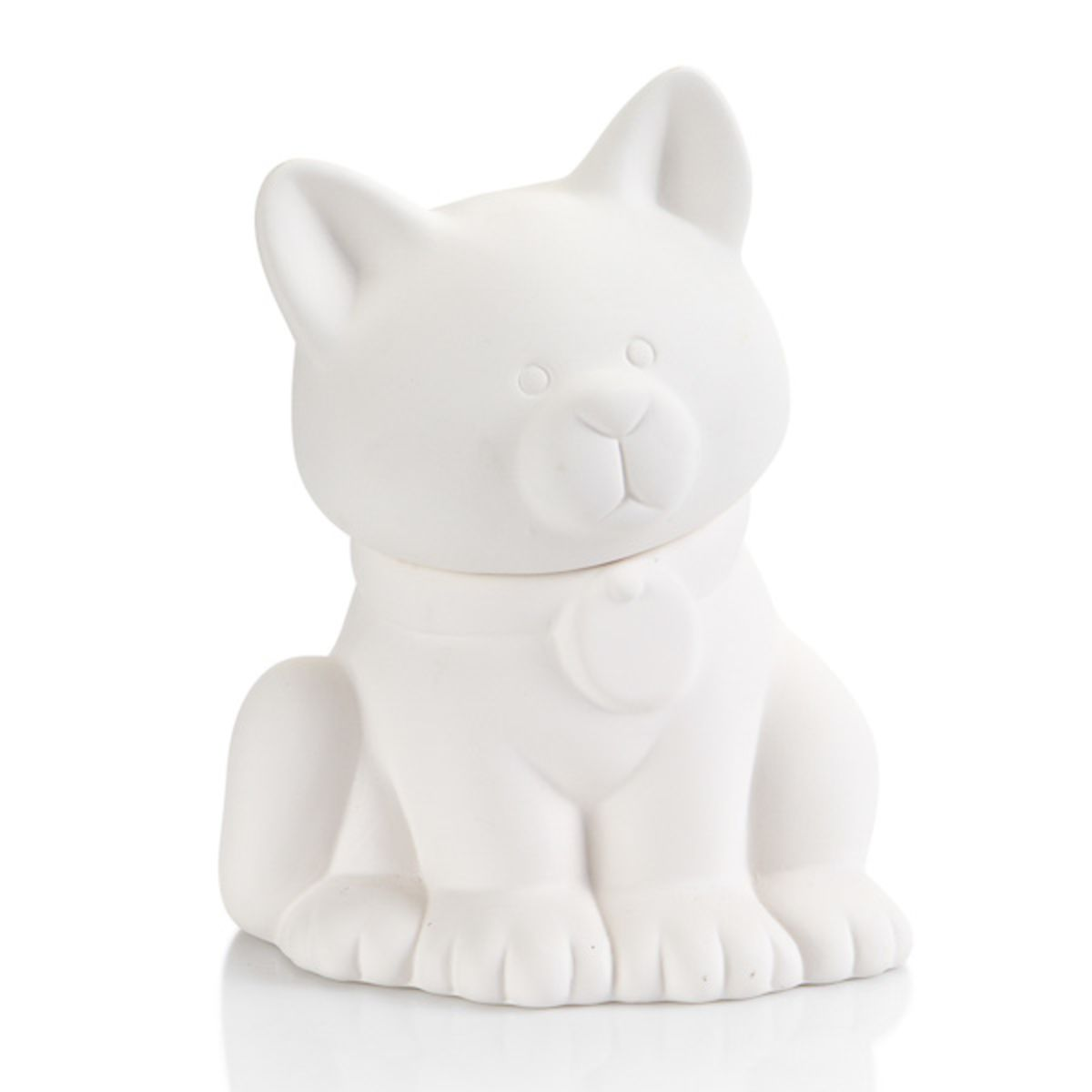(Max of 1)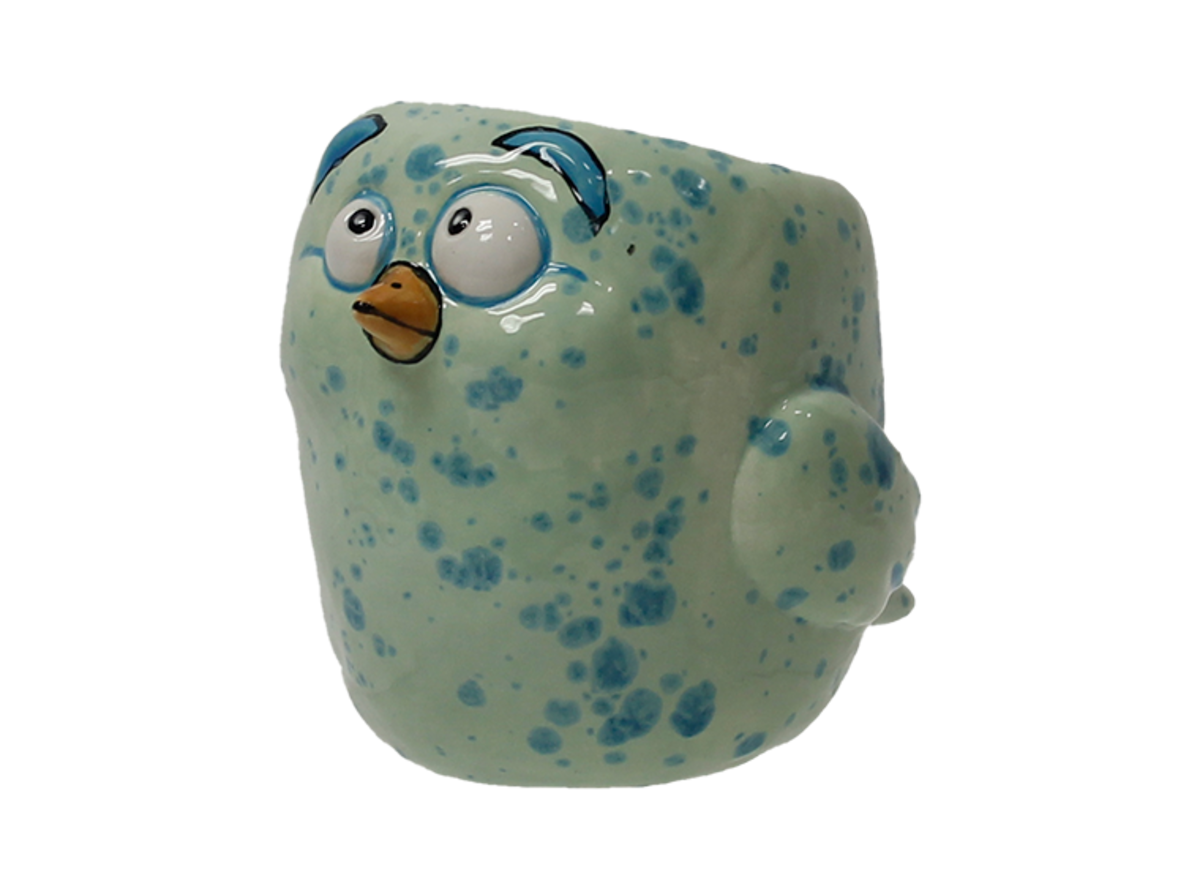(Max of 1)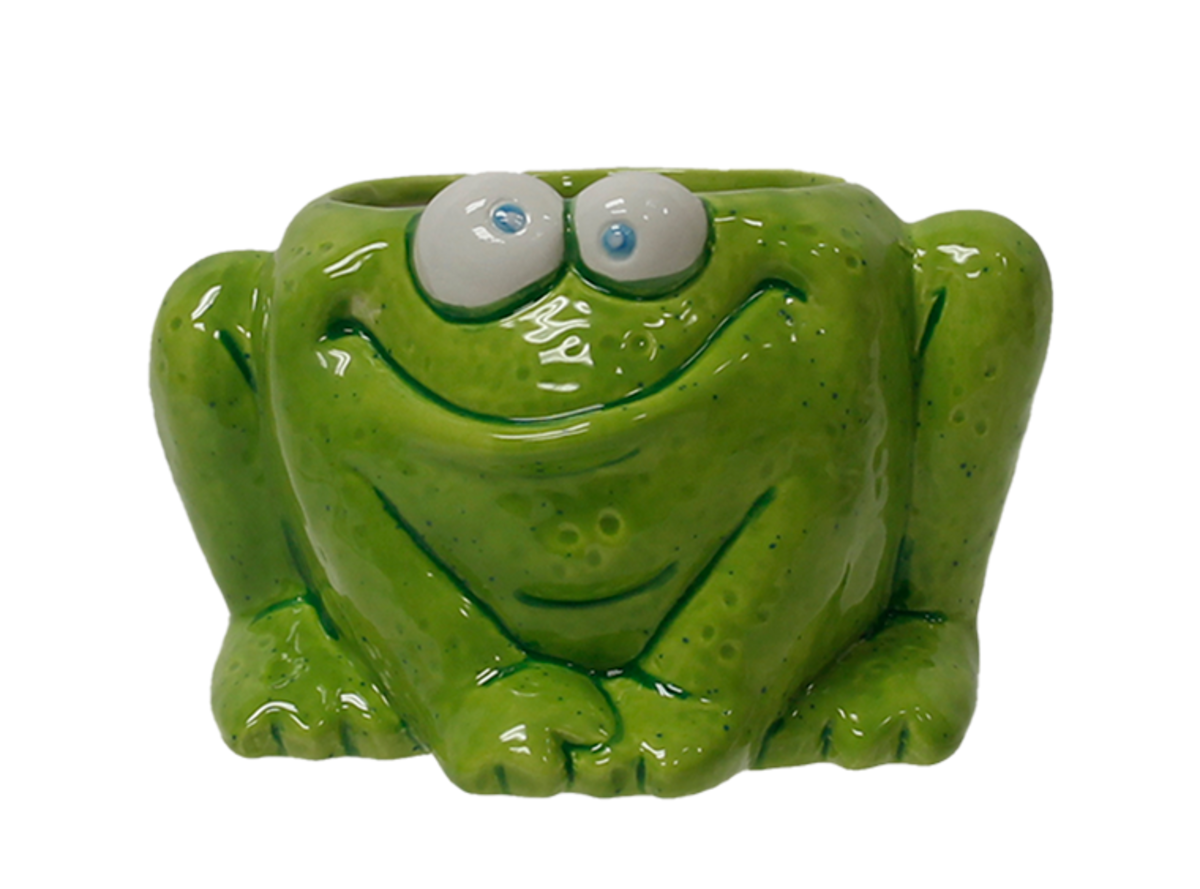(Max of 3)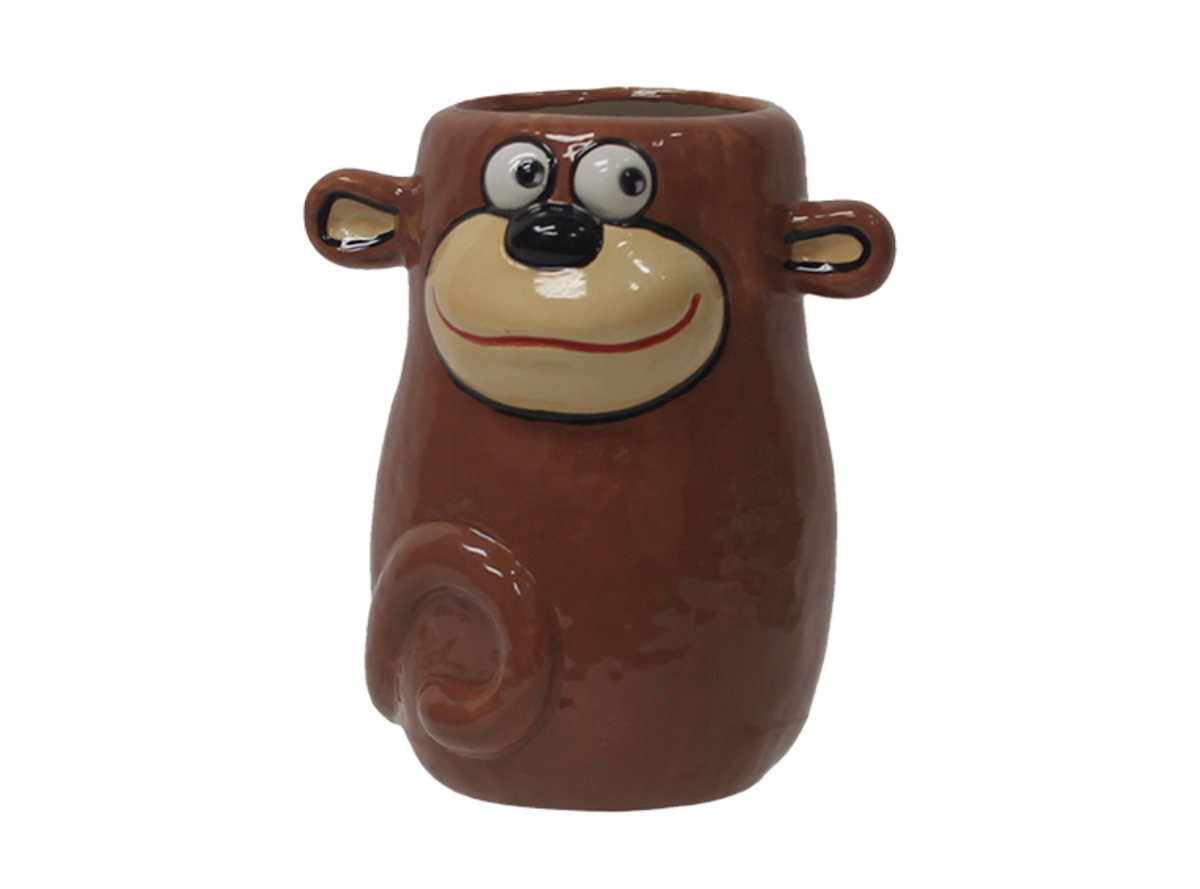(Max of 4)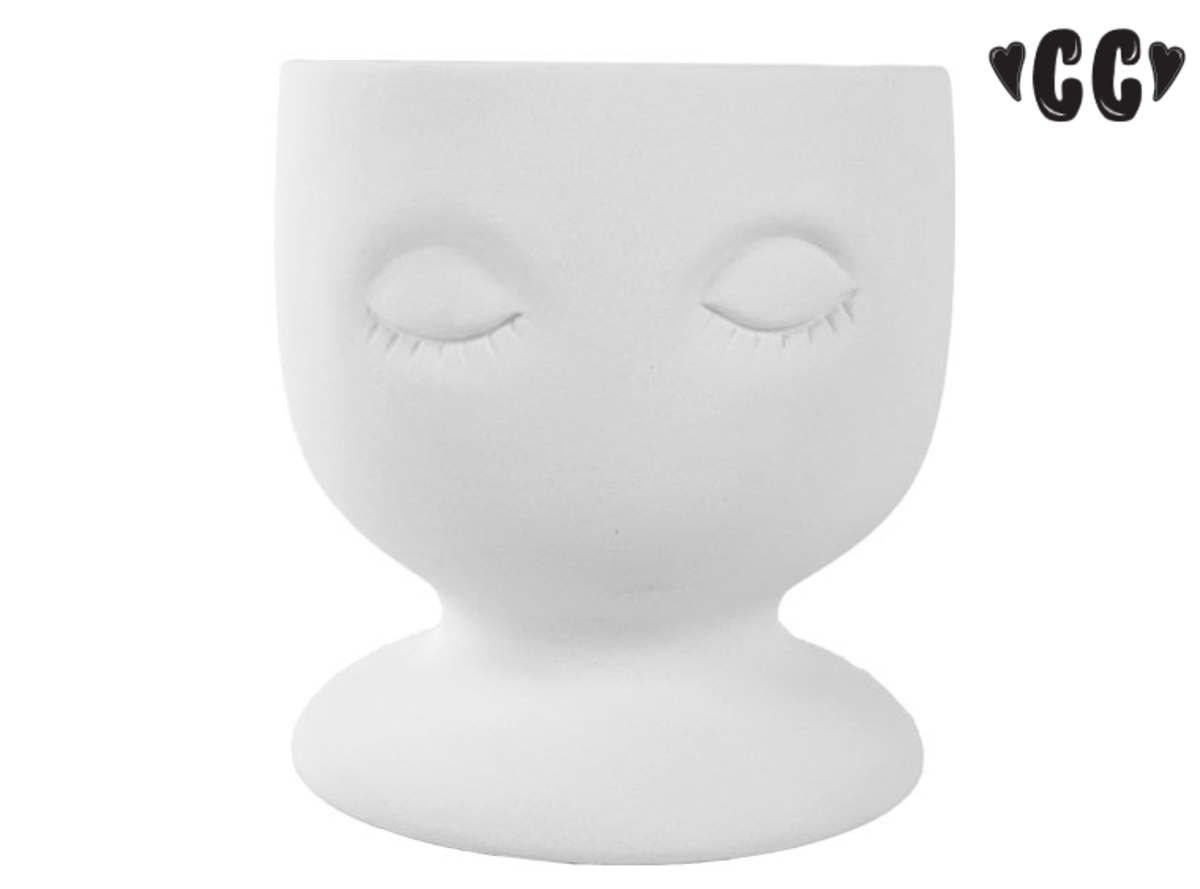(Max of 4)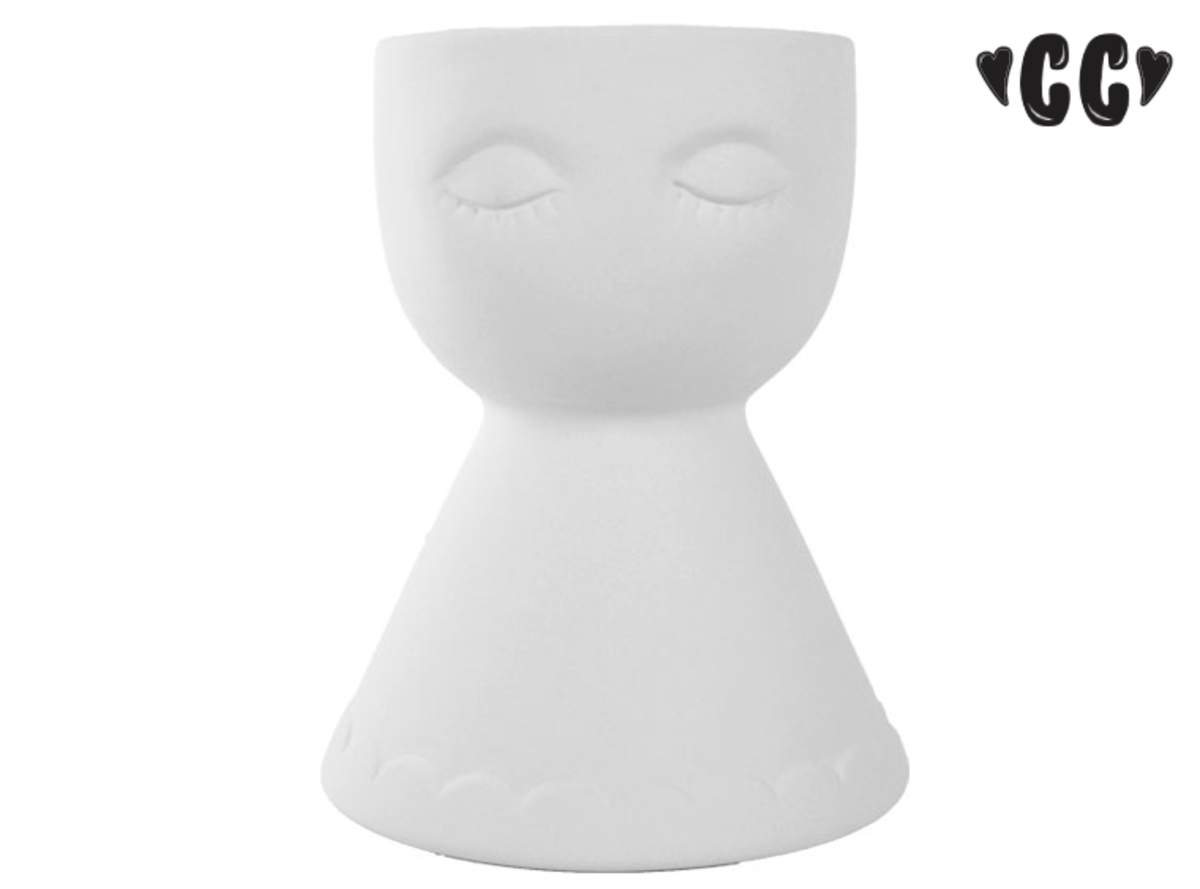(Max of 4)(Max of 3)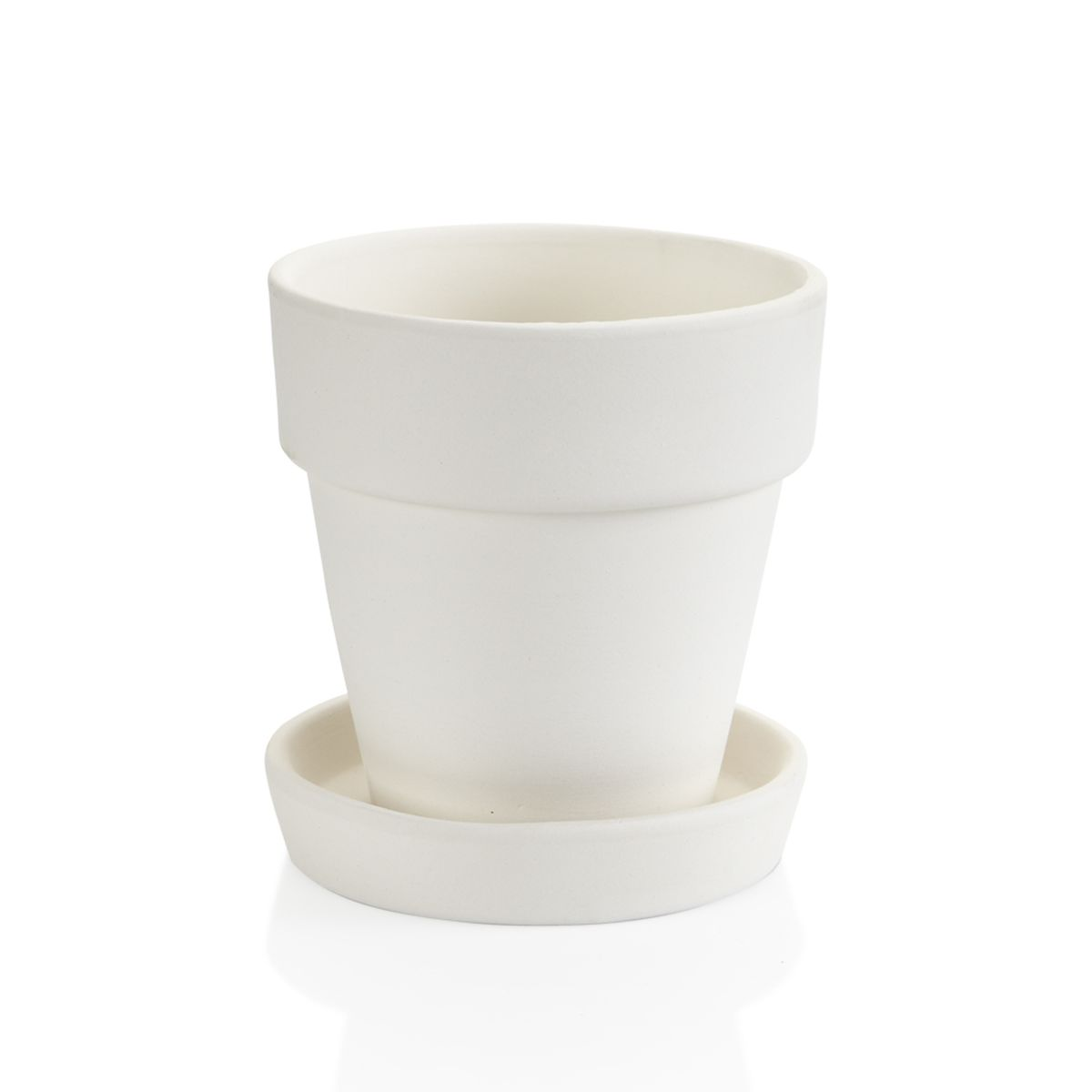(Max of 4)

### Kitchen Items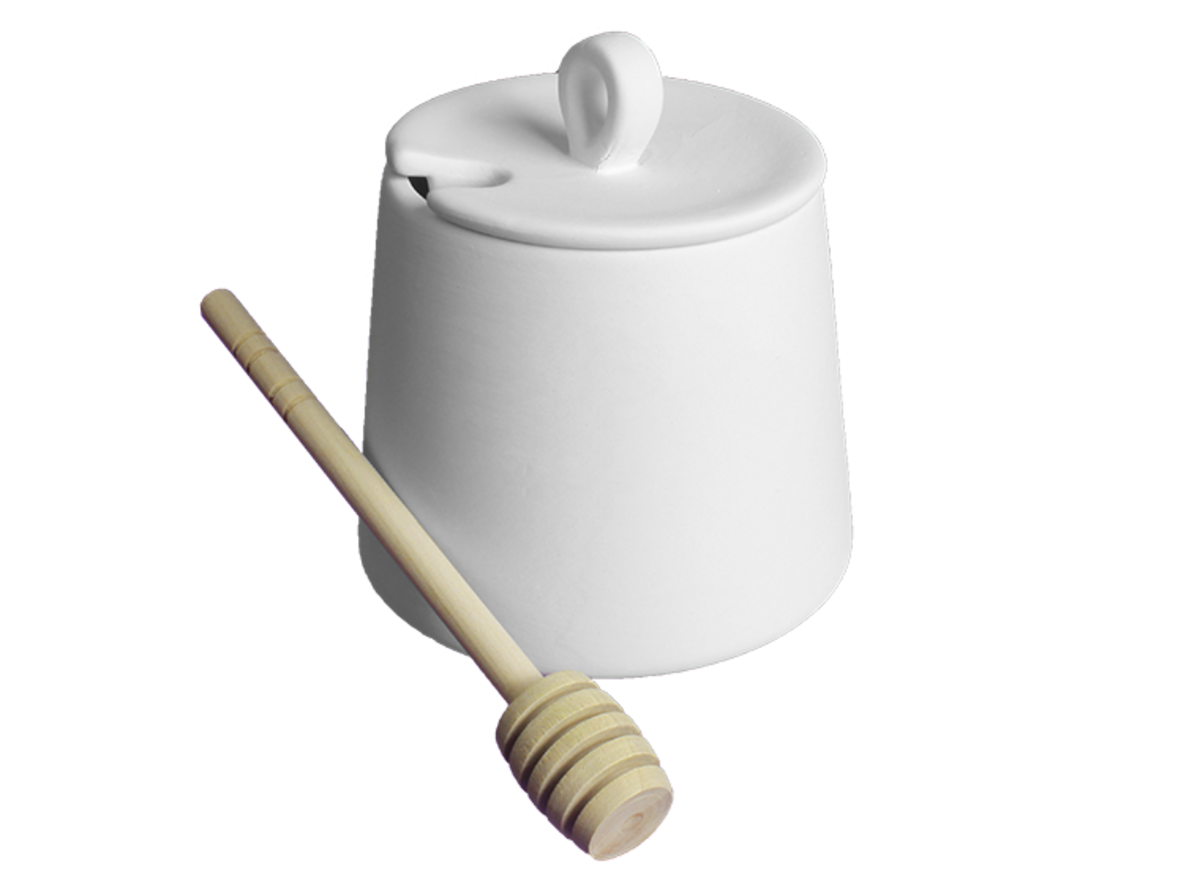(Max of 5)

### Mugs/Sets/Pitchers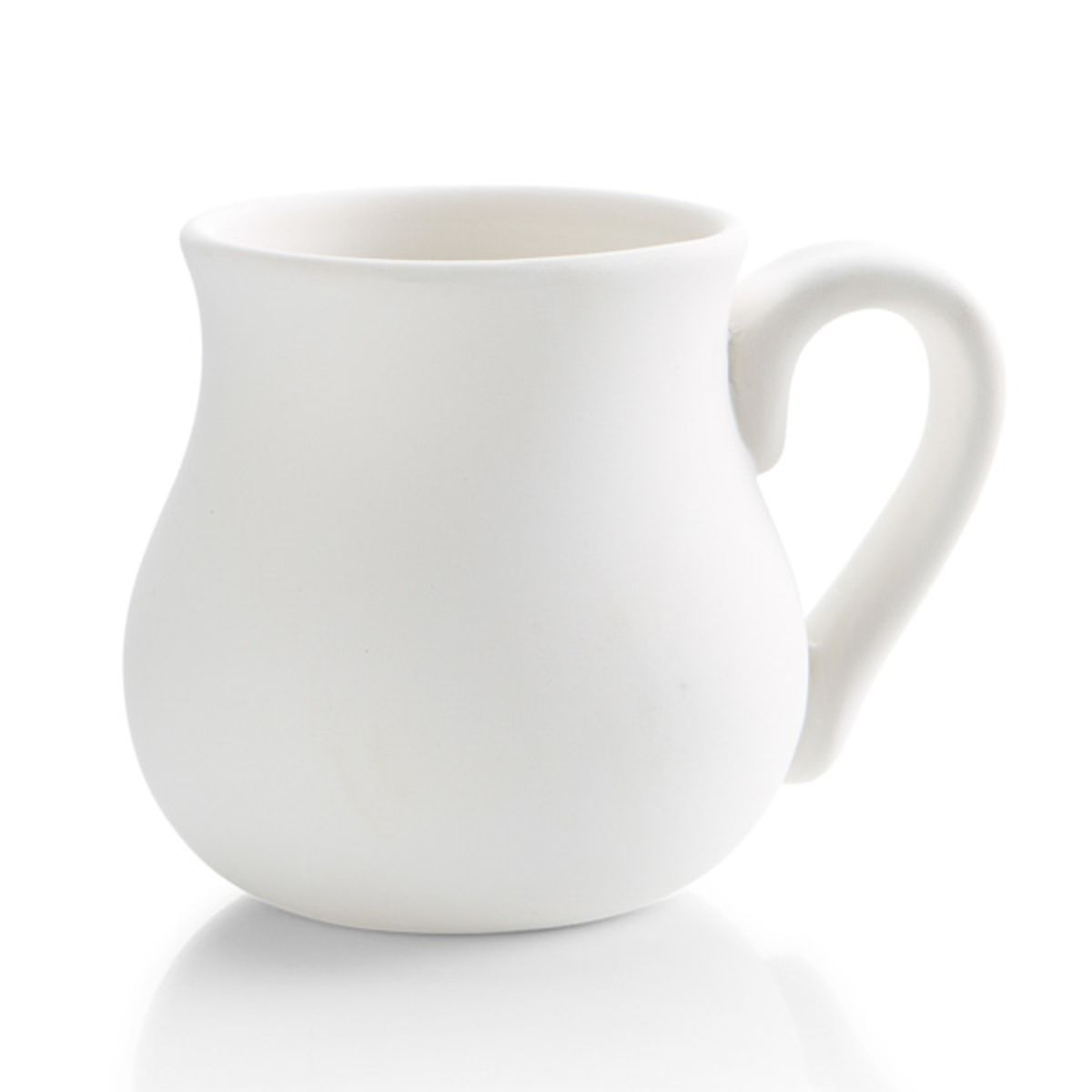(Max of 2)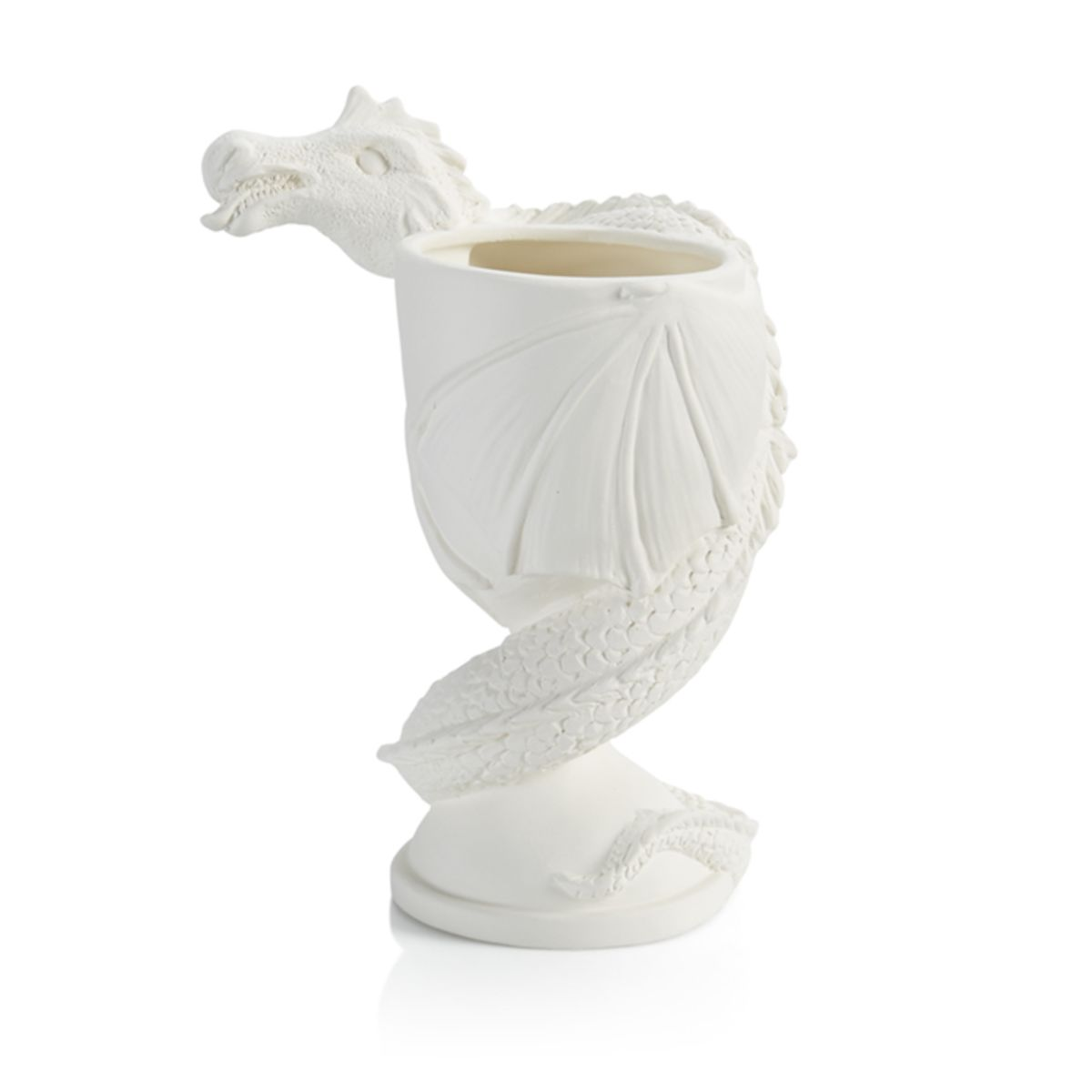(Max of 1)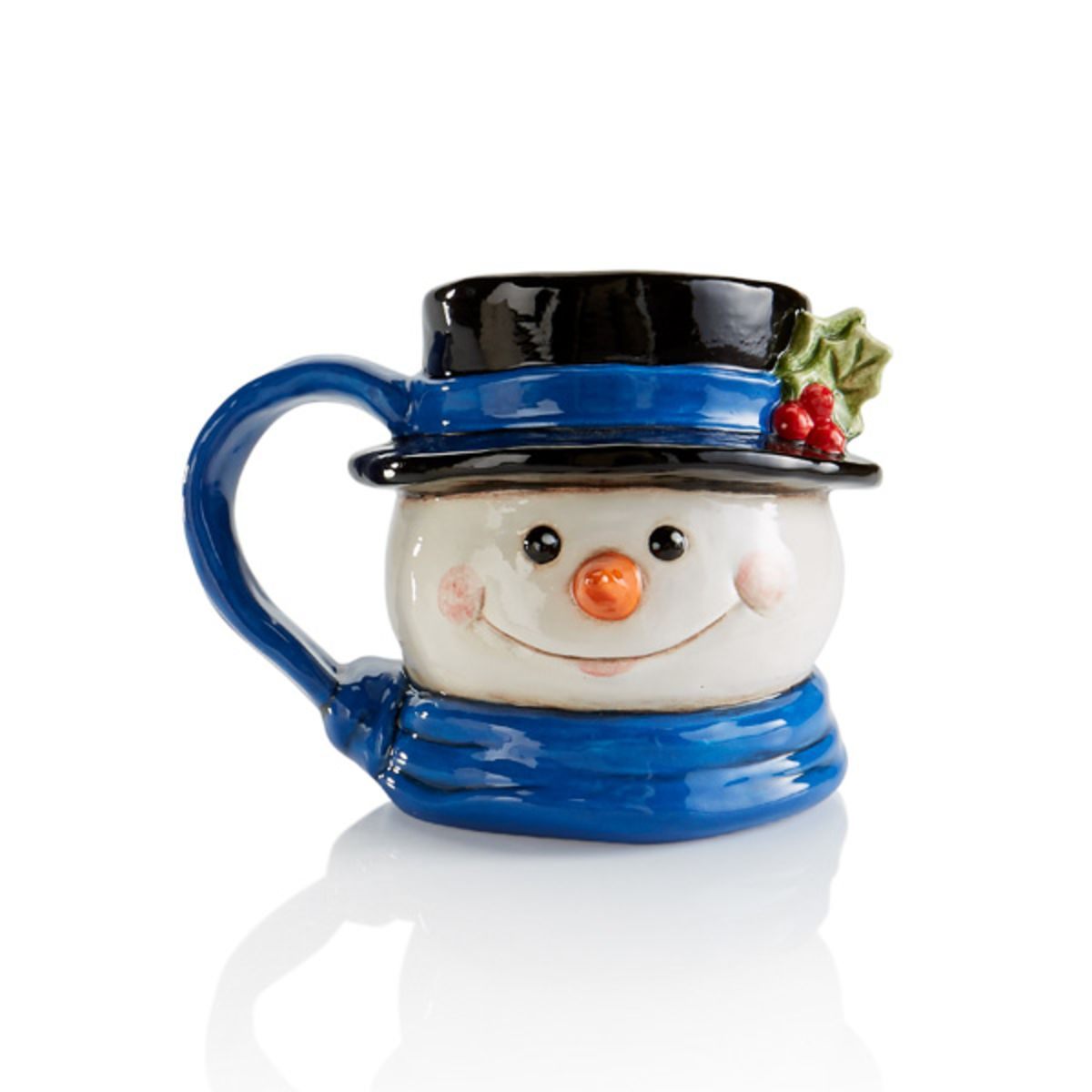(Max of 2)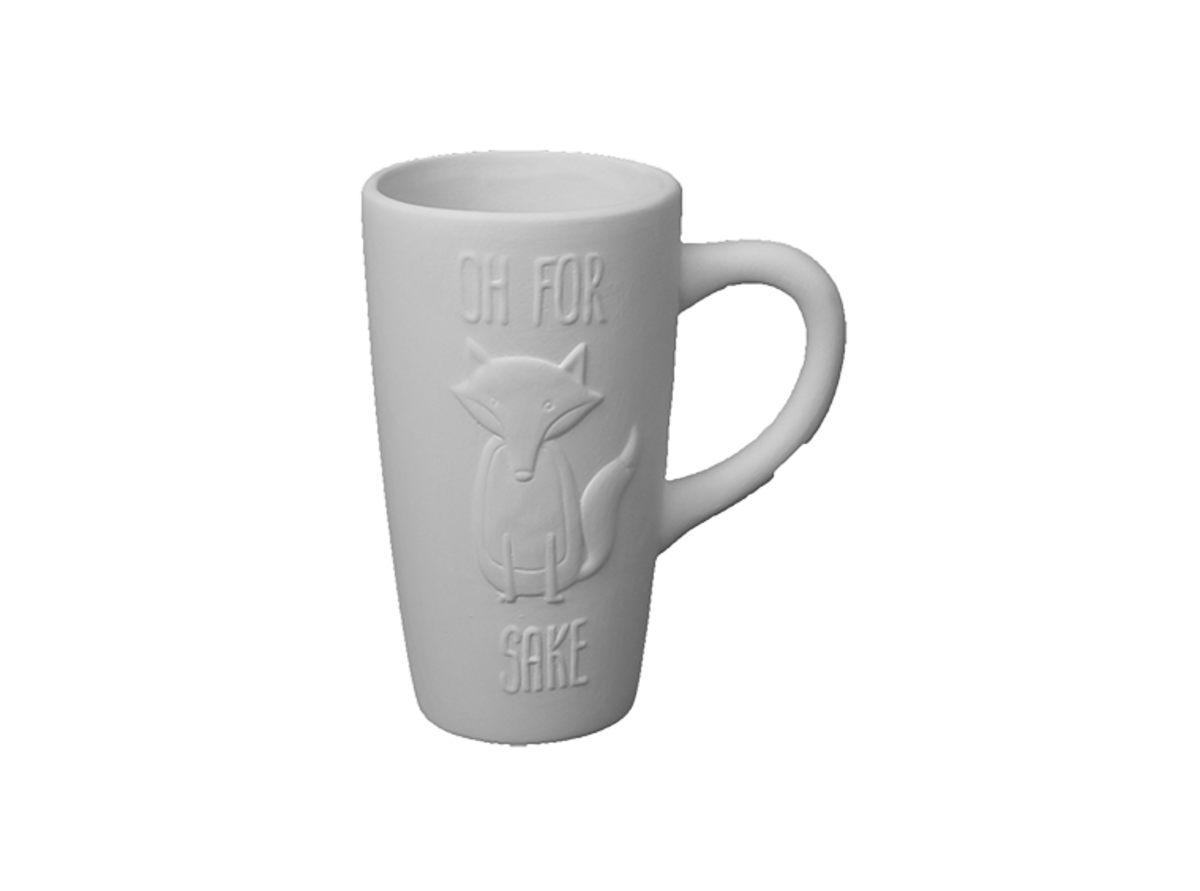(Max of 3)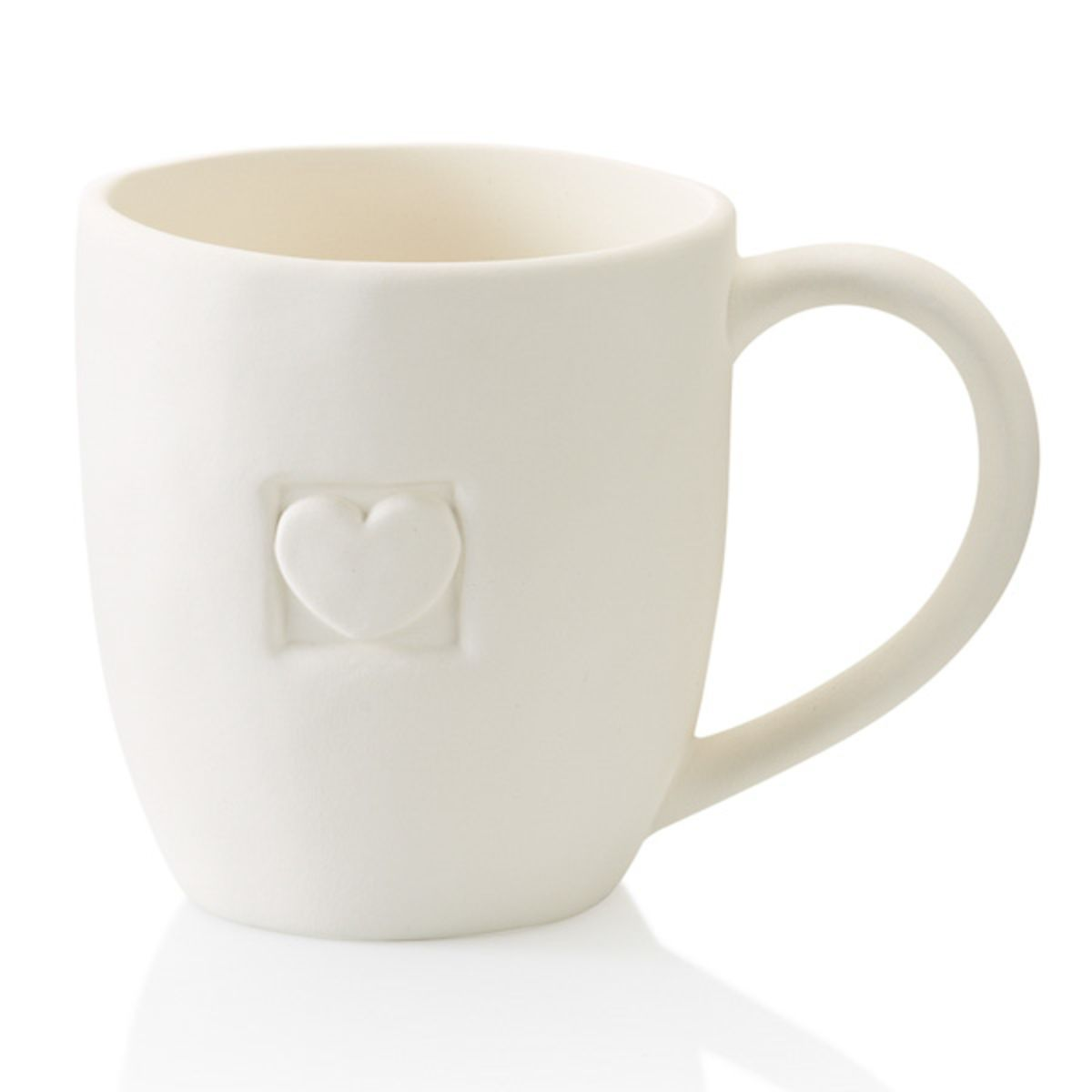(Max of 2)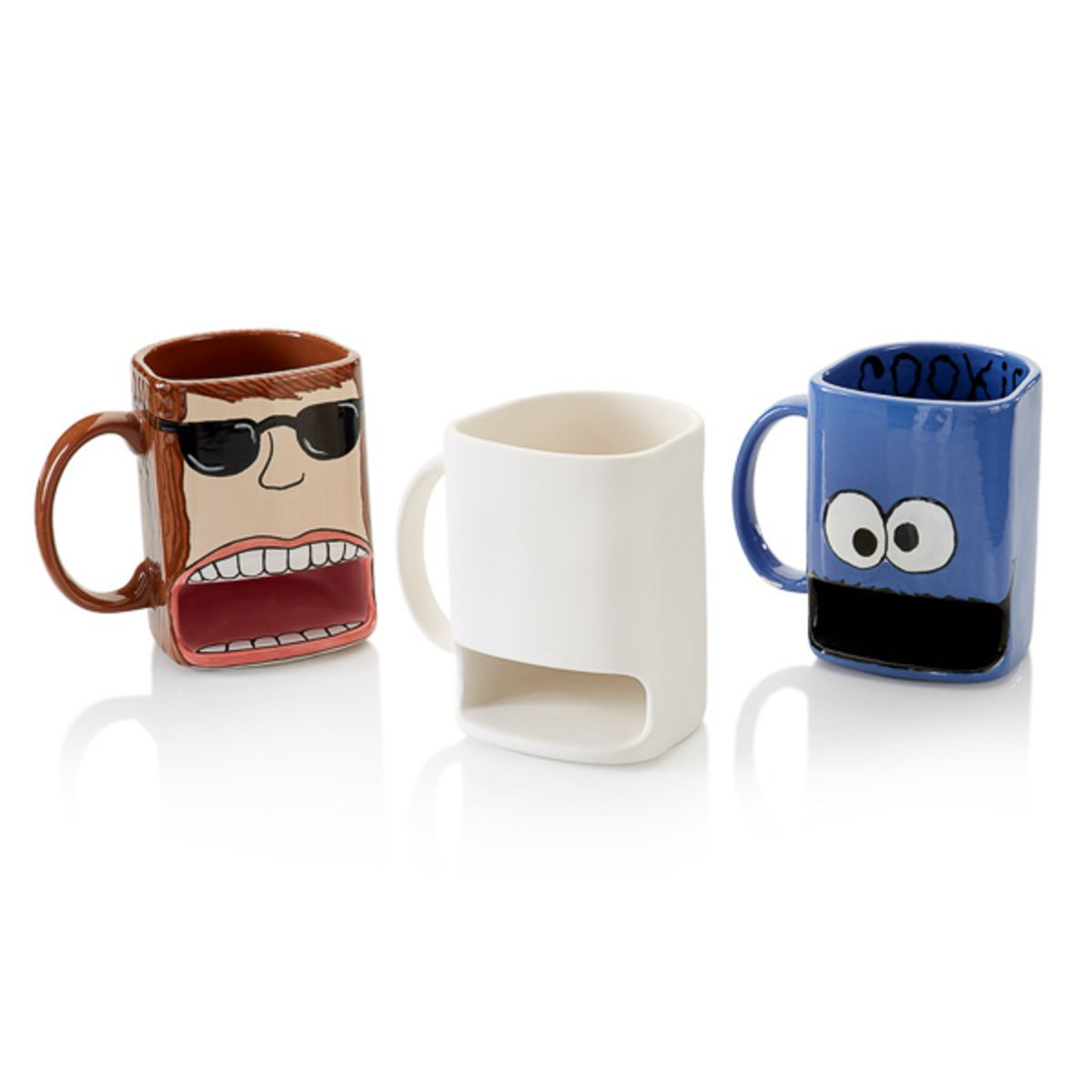(Max of 3)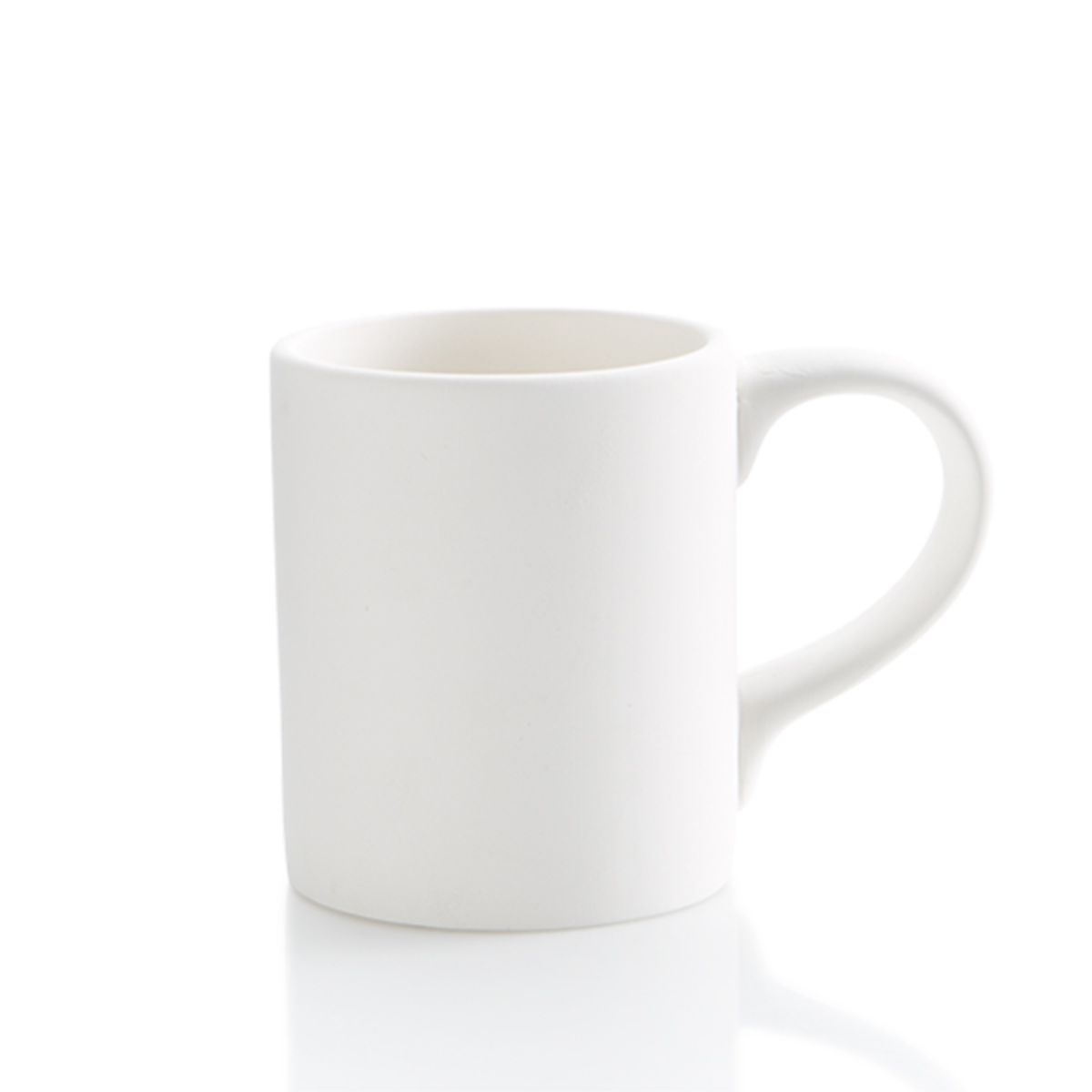(Max of 2)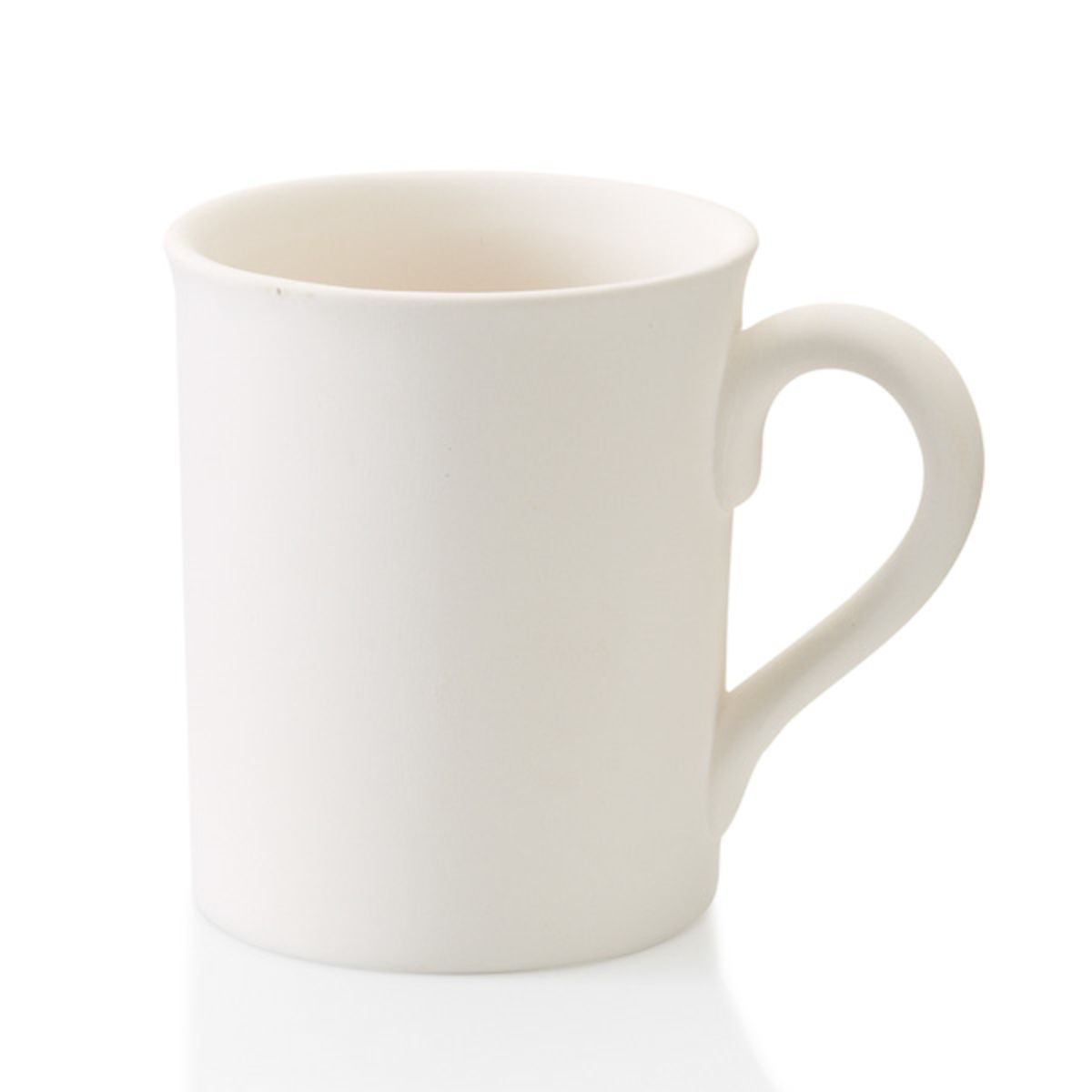(Max of 8)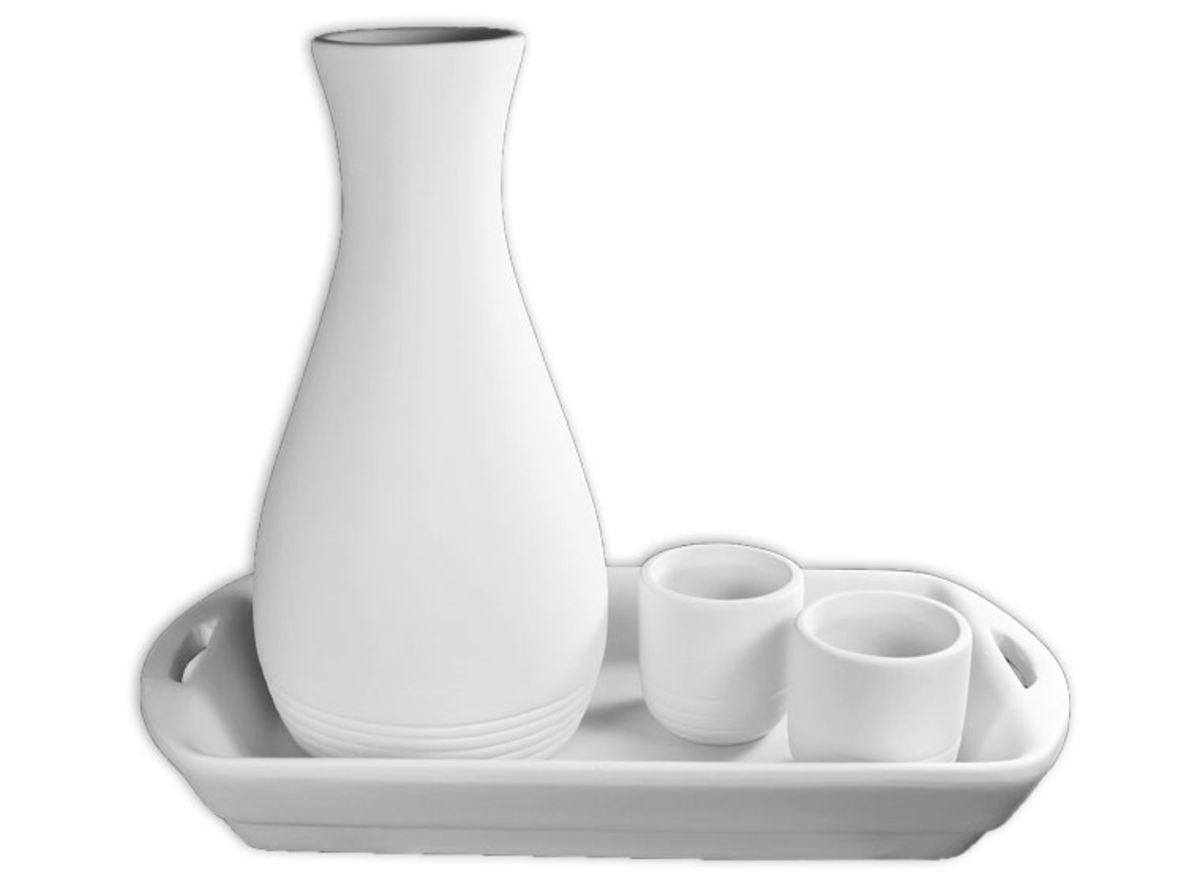(Max of 1)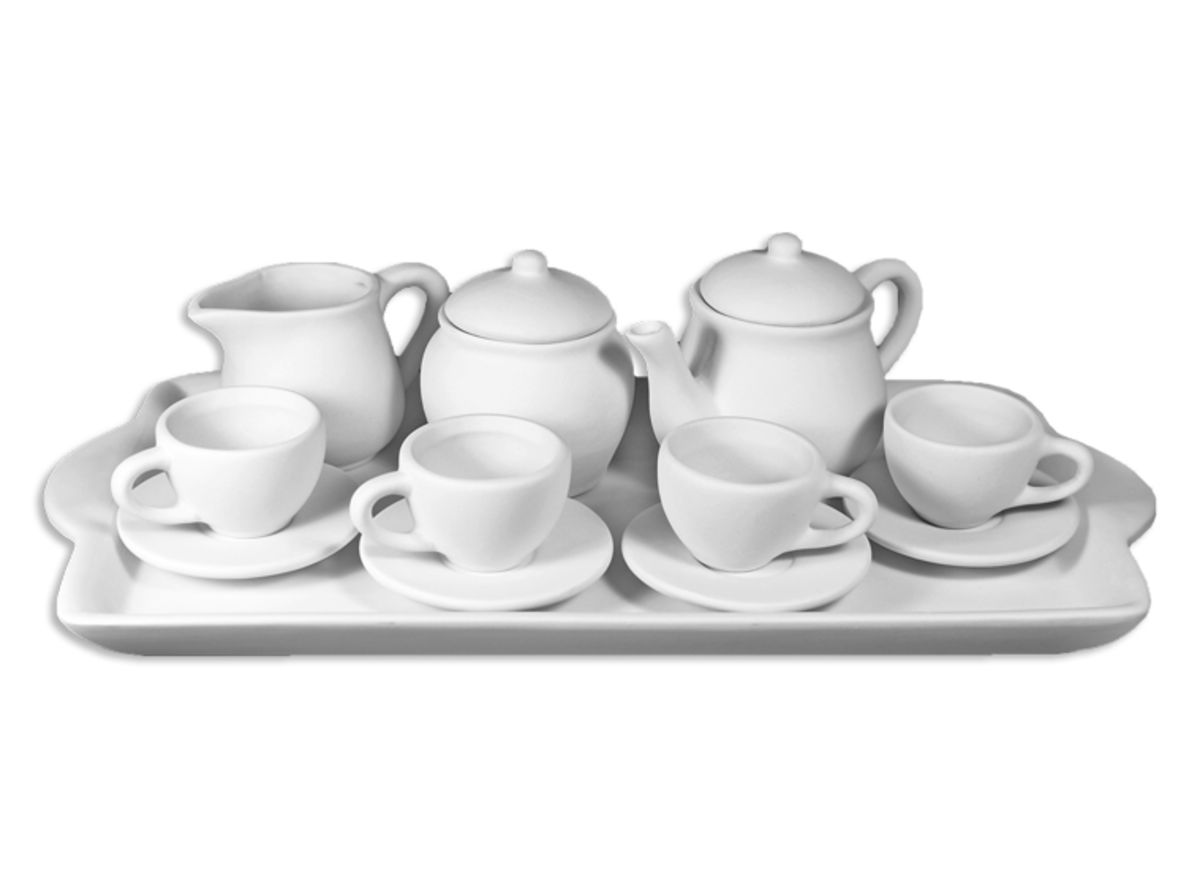(Max of 3)(Max of 11)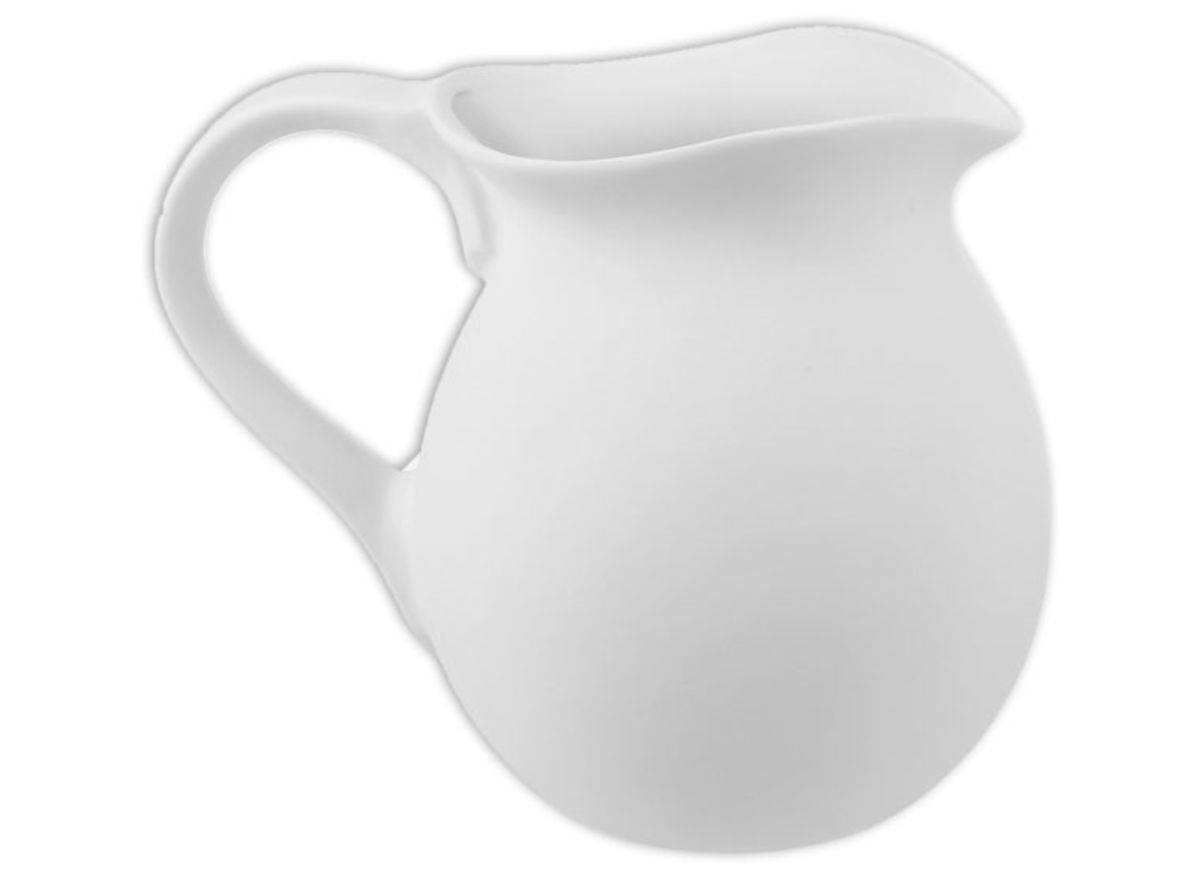(Max of 1)

### Plates/Bowls/Platters(Max of 9)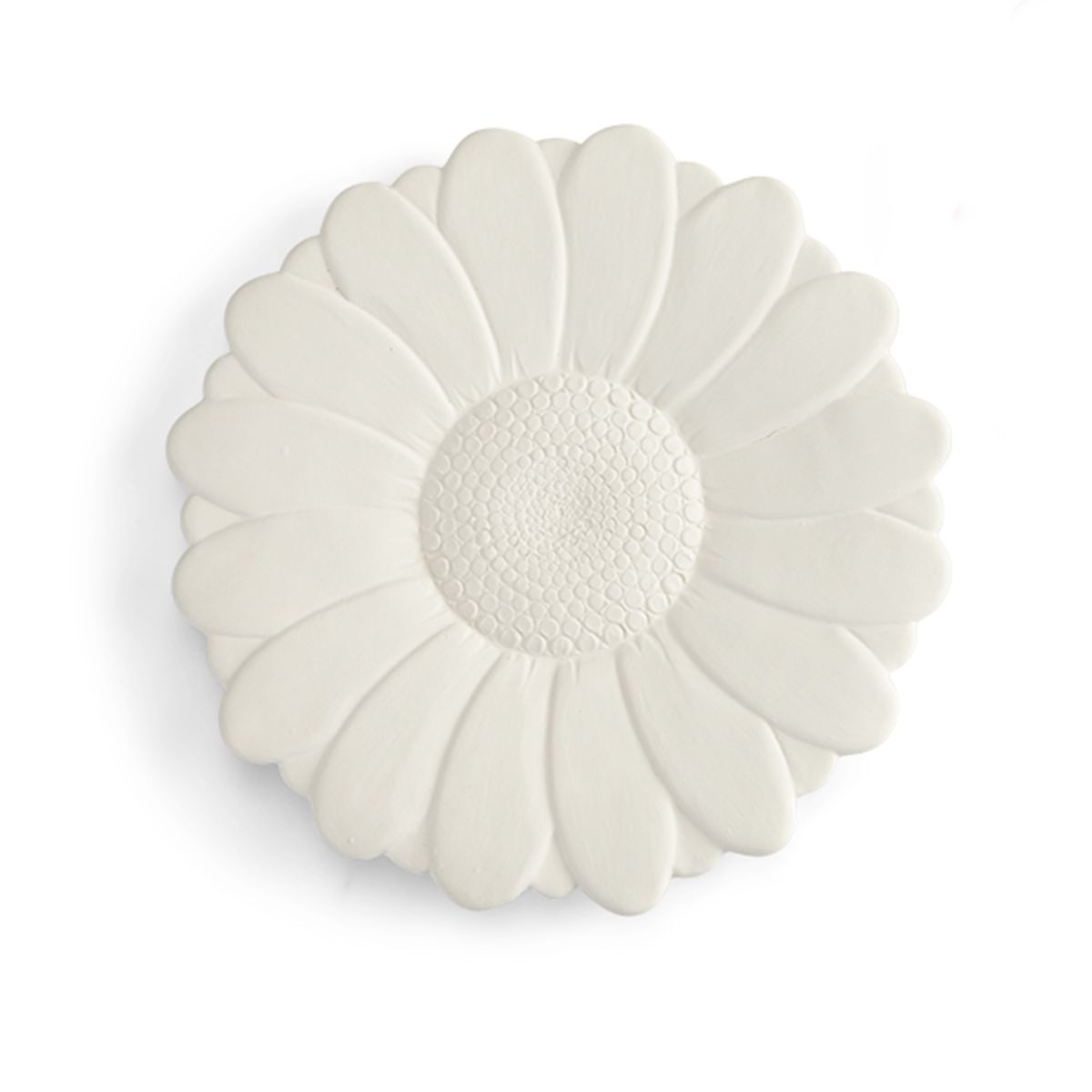(Max of 2)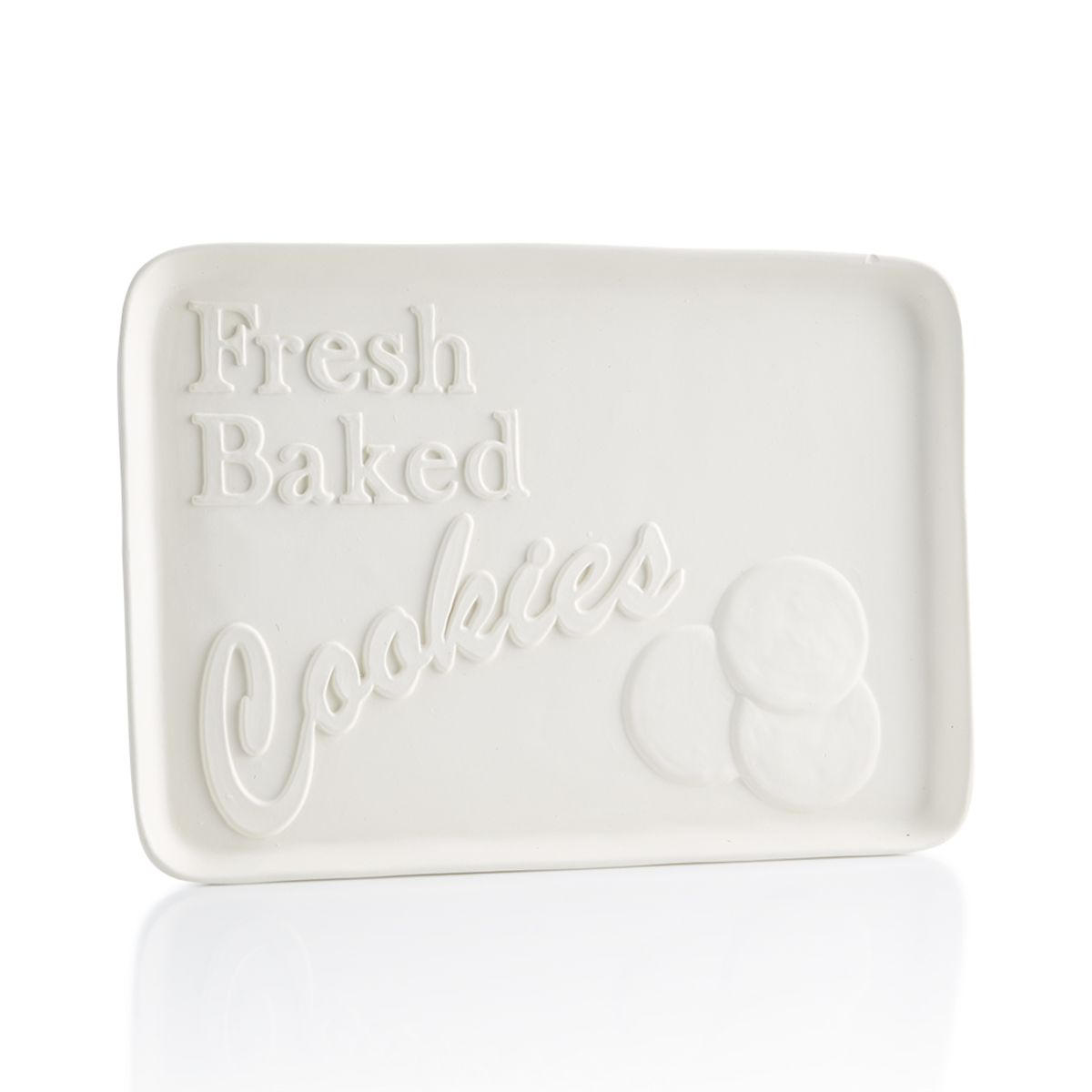(Max of 5)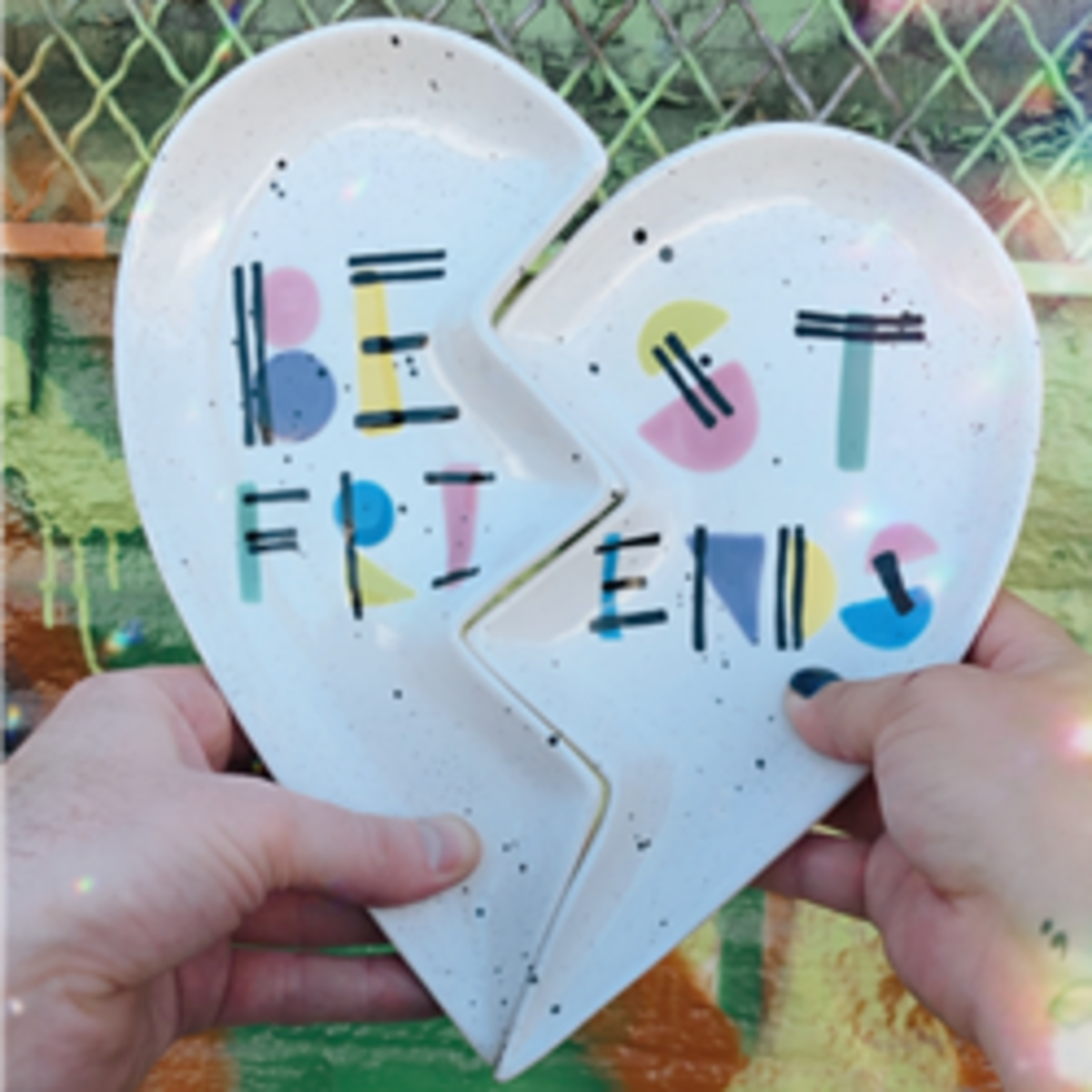(Max of 5)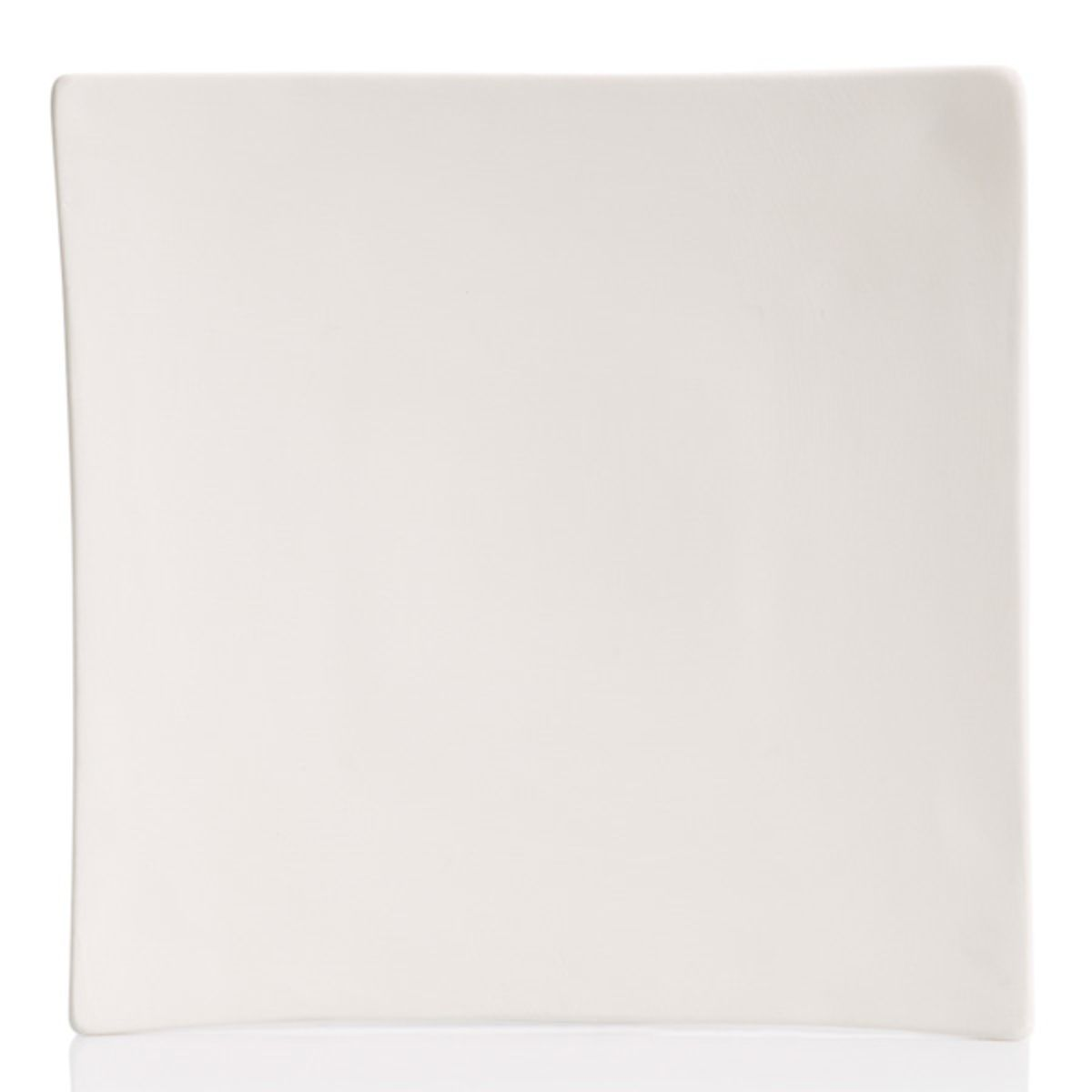(Max of 2)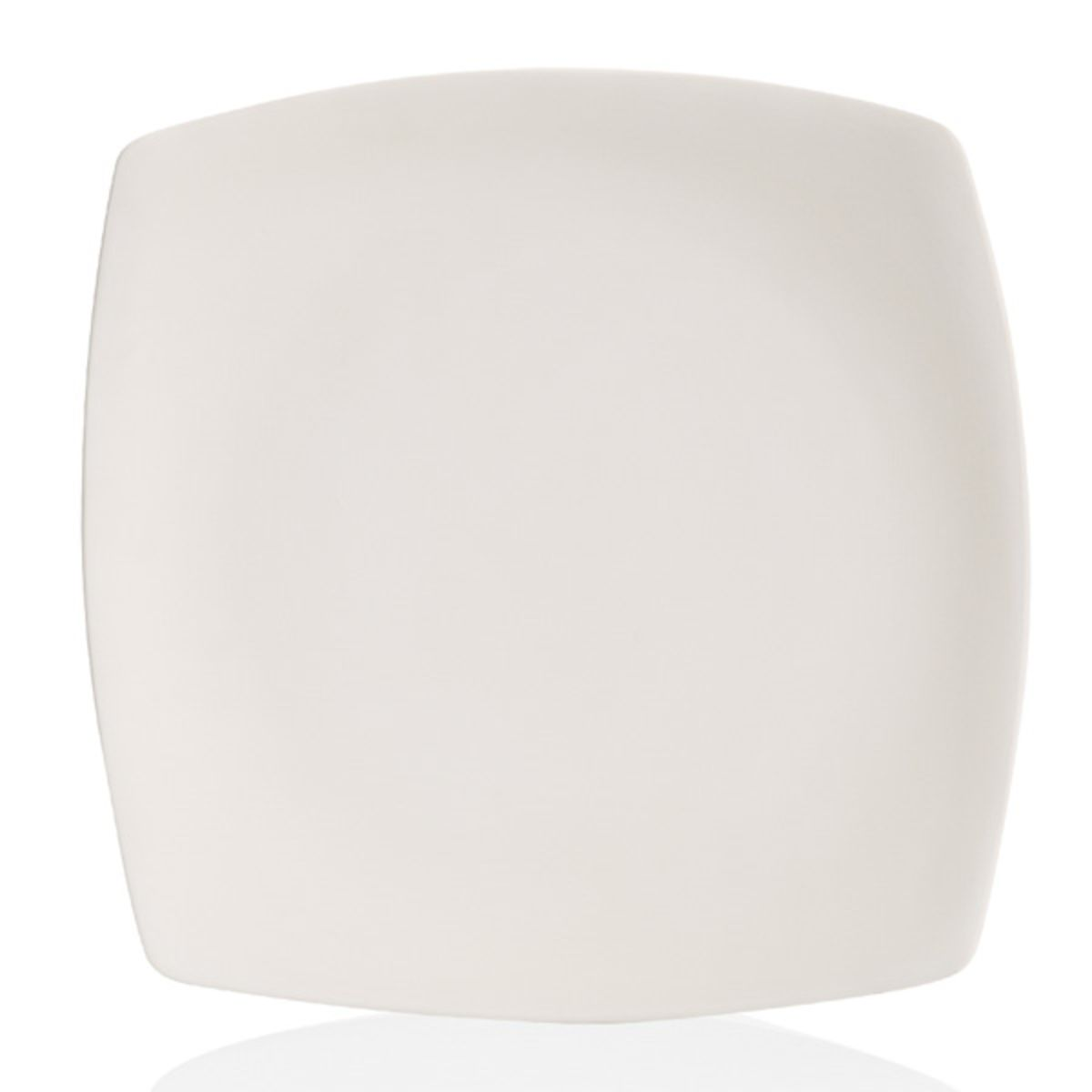(Max of 1)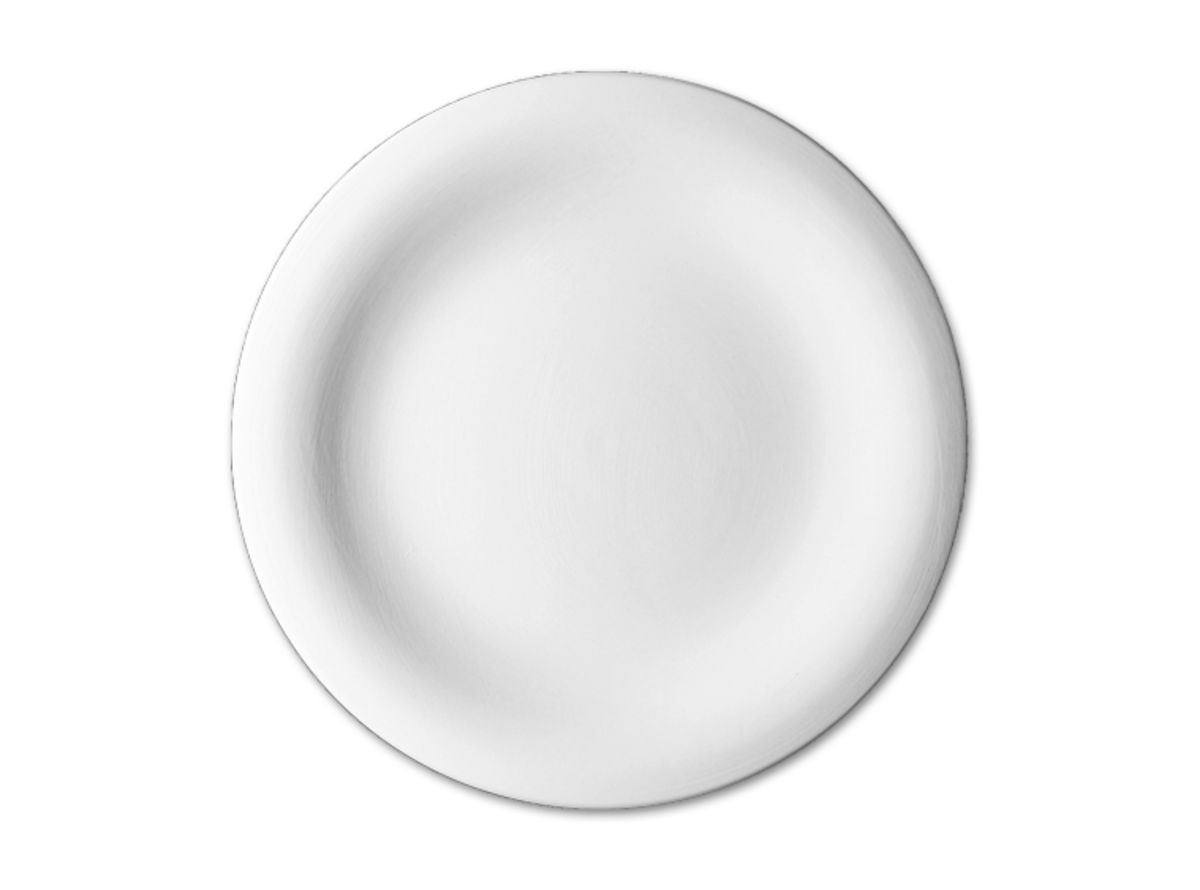(Max of 5)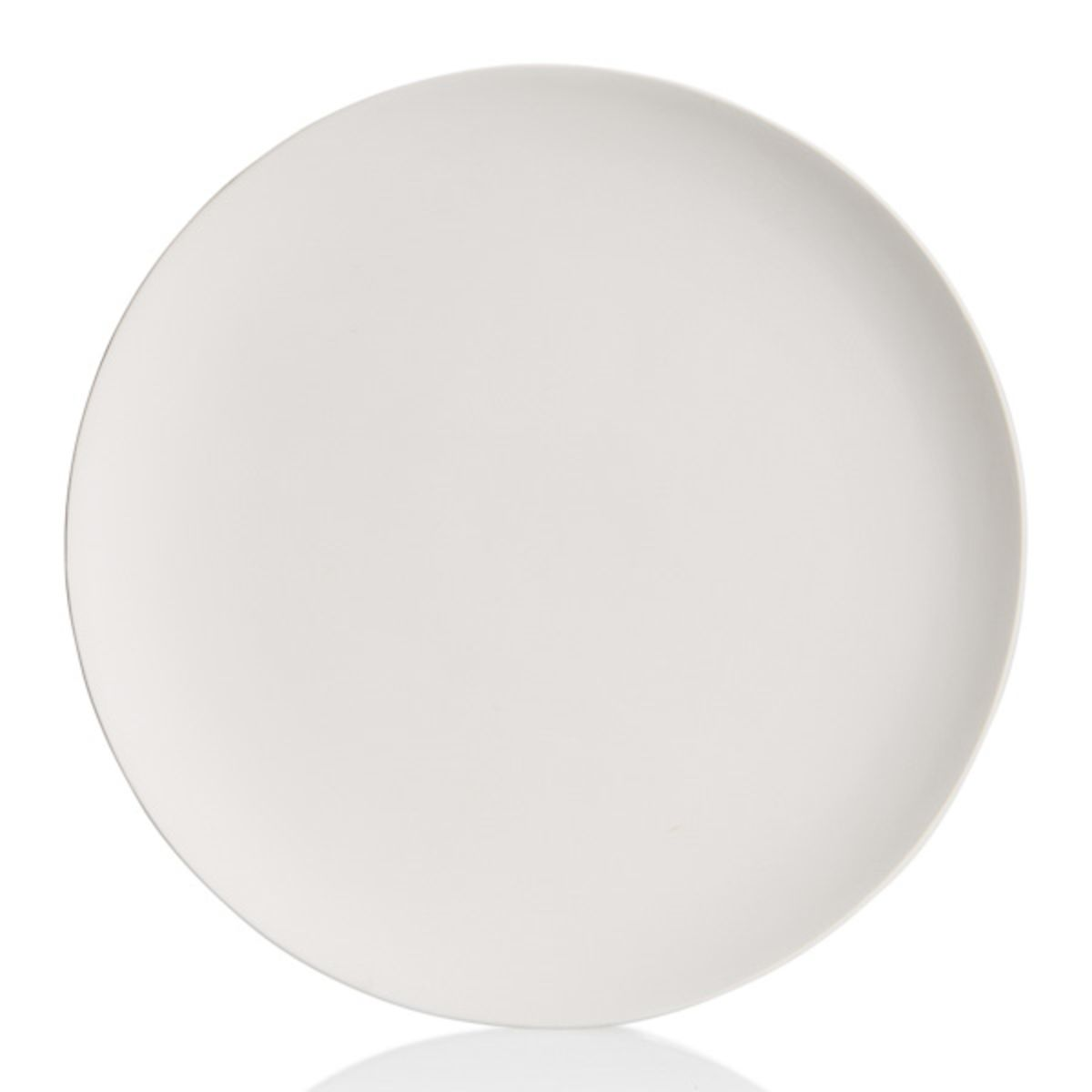(Max of 6)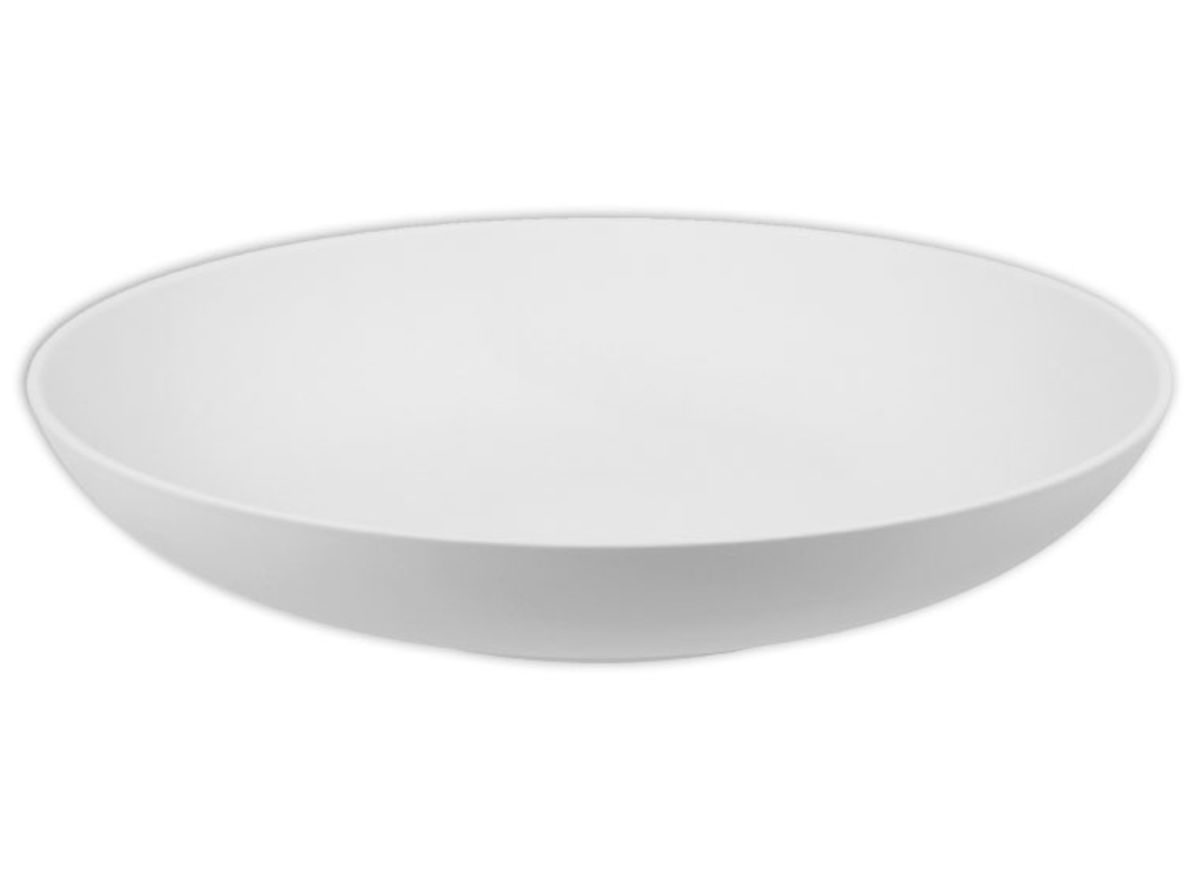(Max of 1)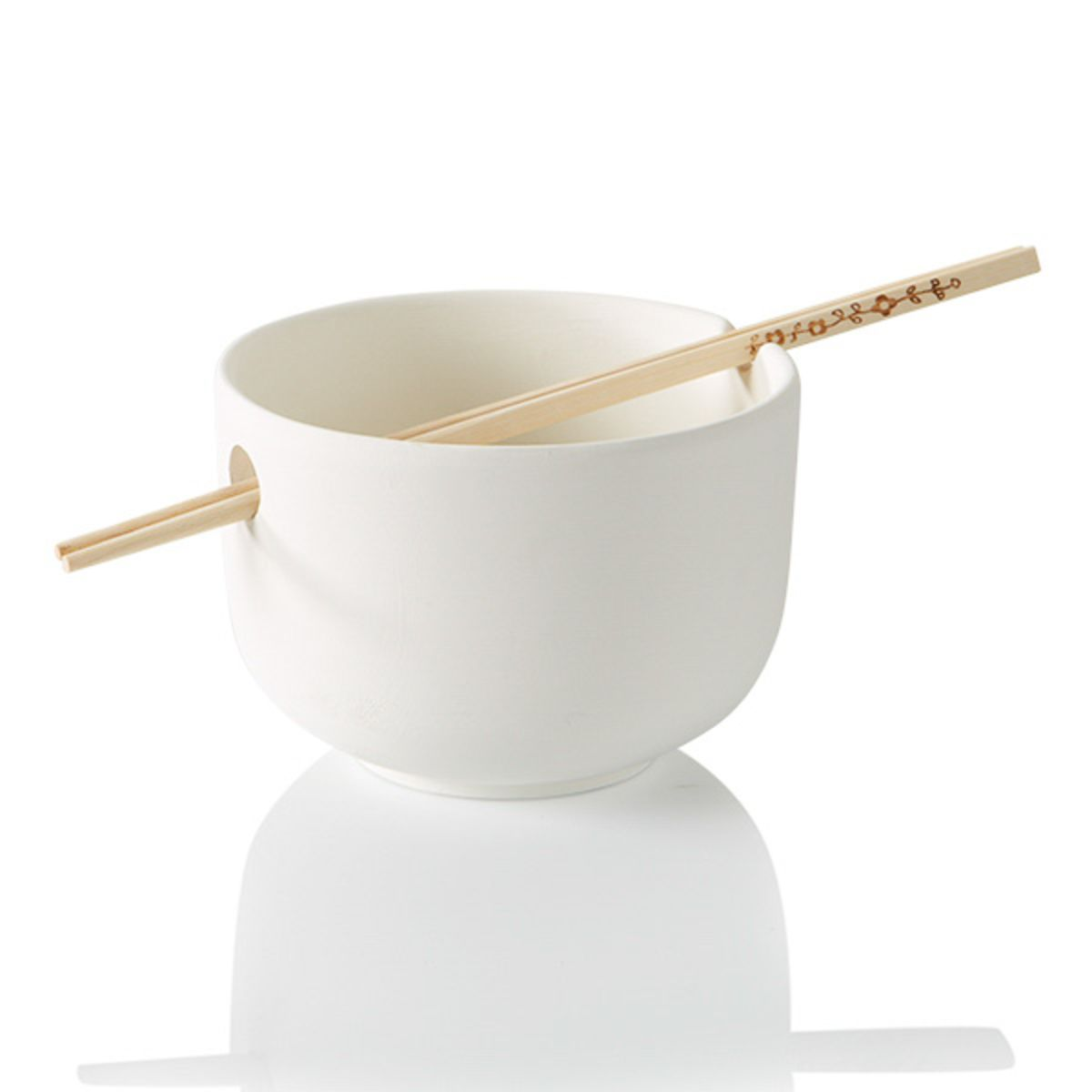(Max of 3)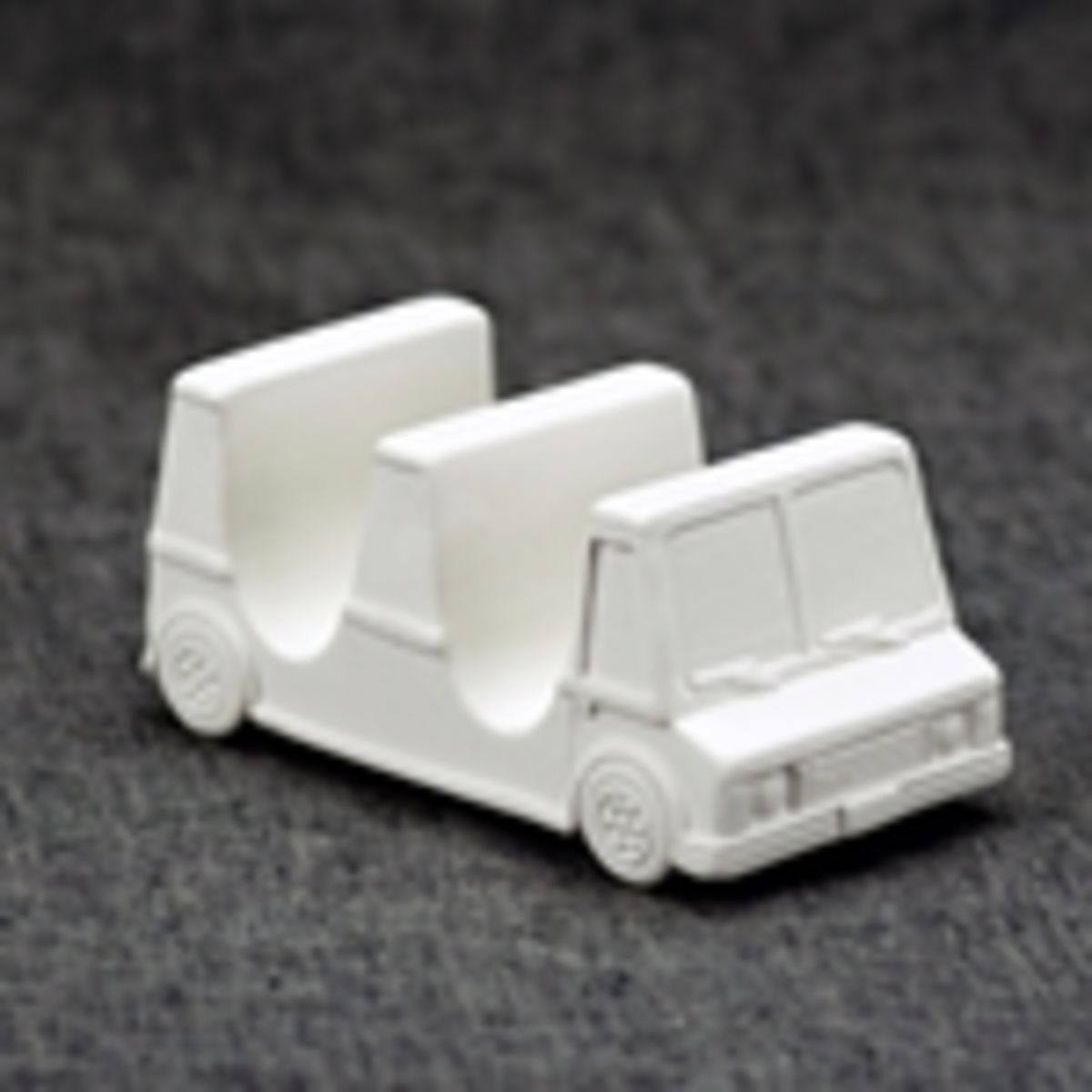(Max of 10)